# WO2010073977A1 - Encoding method, decoding method, apparatus, program, and recording medium therefor - Google Patents

Encoding method, decoding method, apparatus, program, and recording medium therefor Download PDF

## Info

Publication number
WO2010073977A1
WO2010073977A1 PCT/JP2009/071100 JP2009071100W WO2010073977A1 WO 2010073977 A1 WO2010073977 A1 WO 2010073977A1 JP 2009071100 W JP2009071100 W JP 2009071100W WO 2010073977 A1 WO2010073977 A1 WO 2010073977A1
Authority
WO
WIPO (PCT)
Prior art keywords
value
order
coefficient
parcor
coefficients
Prior art date
Application number
PCT/JP2009/071100
Other languages
French (fr)
Japanese (ja)
Inventor

Original Assignee

Priority date (The priority date is an assumption and is not a legal conclusion. Google has not performed a legal analysis and makes no representation as to the accuracy of the date listed.)
Filing date
Publication date
Priority to JP2008325286 priority Critical
Priority to JP2008-325286 priority
Application filed by 日本電信電話株式会社 filed Critical 日本電信電話株式会社
Publication of WO2010073977A1 publication Critical patent/WO2010073977A1/en

## Images

•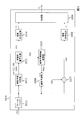•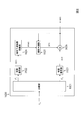•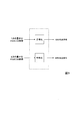•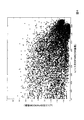•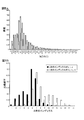•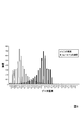•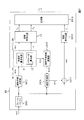•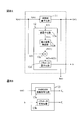••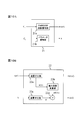•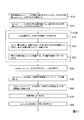•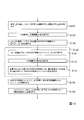•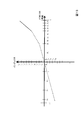•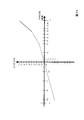•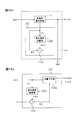•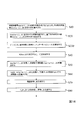•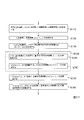•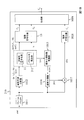•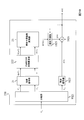•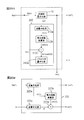••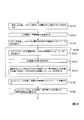•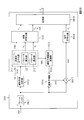•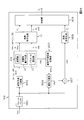•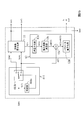•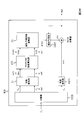••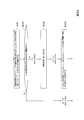•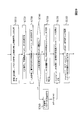•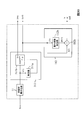•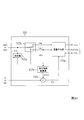•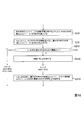•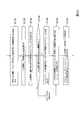•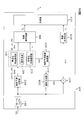•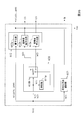••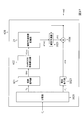•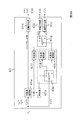•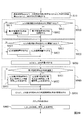•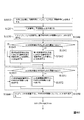## Classifications

• GPHYSICS
• G10MUSICAL INSTRUMENTS; ACOUSTICS
• G10LSPEECH ANALYSIS OR SYNTHESIS; SPEECH RECOGNITION; SPEECH OR VOICE PROCESSING; SPEECH OR AUDIO CODING OR DECODING
• G10L19/00Speech or audio signals analysis-synthesis techniques for redundancy reduction, e.g. in vocoders; Coding or decoding of speech or audio signals, using source filter models or psychoacoustic analysis
• G10L19/04Speech or audio signals analysis-synthesis techniques for redundancy reduction, e.g. in vocoders; Coding or decoding of speech or audio signals, using source filter models or psychoacoustic analysis using predictive techniques
• G10L19/06Determination or coding of the spectral characteristics, e.g. of the short-term prediction coefficients

## Abstract

At least a first-order PARCOR coefficient and a second-order PARCOR coefficient are respectively calculated by the liner prediction analysis of an input time-series signal; a parameter which is determined in accordance with the relational expression which is established between a value which is determined in accordance with the calculated first-order PARCOR efficient and a value which is determined in accordance with the calculated  second-order PARCOR coefficient; and a code corresponding to information including the parameter and the first-order PARCOR coefficient or second-order PARCOR coefficient is generated.  Upon decoding, the correlation between the first-order PARCOR coefficient and the second-order PARCOR coefficient is used to decode the PARCOR coefficients.

## Description

Encoding method, decoding method, the devices, program and recording medium

The present invention relates to a technique for encoding linear prediction analysis of the time series signals, in particular, the encoding method of PARCOR coefficients obtained by linear prediction analysis, decoding method, the devices, a program, and a recording medium.

Or transmitted by the time-series signal a communication path, such as an audible signal and video information, when recording on the information recording medium, a method of time recorded or transmitted after converting the sequence signal into the compressed code is, the transmission efficiency and recording efficiency it is effective in terms of. Along with increased capacity in recent years broadband penetration and storage device prioritizes height of compression than the lossy compression coding system, the lossless compression encoding scheme with complete regeneration conditions of the original signal are emphasized while some (e.g., see non-Patent Document 1). In such circumstances, a technique for lossless compression encoding of acoustic signal using element technology of the linear predictive analysis or the like has been approved as an MPEG (Moving Picture Expert Group) international standard "MPEG-4 ALS" (for example, non-Patent Document 2).

Figure 1 is a block diagram for illustrating a functional configuration of an encoding device 1010 of the conventional lossless compression encoding method. Figure 2 is a block diagram for illustrating a functional configuration of a decoding device 1020 for decoding the code generated by the encoding device 1010 of FIG. Figure 3 is a diagram for the encoding device 1010 of FIG. 1 and the first-order PARCOR coefficient and secondary PARCOR describing how to be encoded. First, the conventional lossless compression encoding method with reference to these drawings.

[Encoding method]
The frame buffer 1011 of the coding apparatus 1010, sampling and quantized PCM (index indicating the n discrete time) (pulse code modulation) the time-series signal x (n) of the form is input. Frame buffer 1011, a predetermined time interval (hereinafter, referred to as "frame") time-series signal x min (n) (n = 1, ..., N) (N is a positive integer) the buffers the encoding device 1010, time-series signal x (n) (n = 1, ..., n) for each frame for encoding.

First, the time-series signal x (n) for one frame (n = 1, ..., N) is sent to the linear prediction analyzer 1012, the linear prediction analyzer 1012, M order from the primary by the linear prediction analysis PARCOR coefficients up k (m) (m = 1,2, ..., M) is calculated. Incidentally, M is a positive integer indicating the prediction order. Further, m and the following PARCOR coefficients, means a PARCOR coefficient of the linear prediction model of prediction order m. Further, in the linear prediction analysis, the time-series signal x at a certain point in time n (n), which time point n-1 is also past the M than n, n-2, ..., nM of the time-series signal x ( n-1), x (n-2), ..., coefficient x a (nM), respectively α (m) (m = 1, ..., weighted by M) (referred to as "LPC coefficients") assume and stuff, between the prediction residuals e (n), the linear combination is established. Linear prediction model based on this assumption are as follows. In the linear prediction analysis, sequence signal x (n) when the input (n = 1, ..., N) with respect to the linear prediction coefficients the following α (m) (m = 1,2, ..., M ) or a convertible PARCOR coefficients k (m) (m = 1,2, ..., to calculate the coefficients, such as M). Incidentally, the beta · gamma shows the product beta × gamma between beta and gamma.

e (n) = x (n) + α (1) · x (n-1) + α (2) · x (n-2) + ... + α (M) · x (nM)
Further, the time series signal y (n) at a certain point of time n, the time point n-1 is also past the M than n, n-2, ..., nM of the time-series signal x (n-1), x (n-2), ..., x the formula linear FIR (Finite Impulse Response) filter of estimated using (nM) referred to as "linear prediction filter."

y (n) = - {α (1) · x (n-1) + α (2) · x (n-2) + ... + α (M) · x (nM)}
PARCOR coefficients from the primary calculated to M order k (m) (m = 1,2, ..., M) is sent to the nonlinear quantization section 1013, from the primary to the M-th order quantized quantized PARCOR coefficients i (m) (m = 1,2, ..., M) is generated. Incidentally, "the quantized PARCOR coefficient" may be a quantized value itself PARCOR coefficient may be an index attached to the quantized value of the PARCOR coefficients. Quantization from the primary to M order PARCOR coefficients i (m) (m = 1,2 , ..., M) is sent to the coefficient coding unit 1014, where entropy-coded by the coefficient code C k is It is generated. This coding primary PARCOR coefficient of the M th order from k (m) (m = 1,2, ..., M) is performed independently for each. For example, as shown in FIG. 3, the first-order quantized PARCOR coefficients i (1) and second-order quantized PARCOR coefficients i (2), are entropy coded independently of one another. The quantization from the primary to M order PARCOR coefficients i (m) (m = 1,2, ..., M) is also sent to the linear prediction coefficient conversion unit 1015. Linear prediction coefficient conversion unit 1015, the linear prediction coefficients of the linear prediction filter prediction order M using these α (m) (m = 1,2, ..., M) is calculated. Linear prediction unit 1016, one frame of the time-series signal x (n) (n = 1, ..., N) and the linear prediction coefficient α (m) (m = 1,2, ..., M) using the door, linear prediction value by a linear prediction y (n) (n = 1, ..., n) to generate a. Subtraction unit 1017, when the prediction residual obtained by subtracting the linear prediction values ​​y (n) from the series signal x (n) (sometimes referred to as "prediction error") is calculated e (n) (prediction filtering). Calculated prediction residuals e (n) is sent to residual coding unit 1018, where entropy-coded by residual code C e is generated. A coefficient code C k generated by the coefficient coding section 1014, residual code C e generated by the residual encoding unit 1018 is sent to the synthesis unit 1019. Coefficient code C k and the residual code C e is combined by the combining unit 1019, the code C g is generated.

[Decoding method]
Code C g input to the decoding device 1020 is separated into a coefficient code C k and the residual code C e by the demultiplexer 1021. The coefficient code C k and the residual code C e, is respectively decoded by the coefficient decoding unit 1022 and the residual decoder 1023, a quantization from the primary to M order PARCOR coefficients i (m) (m = 1 , ... , and M), the prediction residuals e (n) (n = 1, ..., n) and is generated. Quantization from the primary to M order PARCOR coefficients i (m) (m = 1, ..., M) is sent to the linear prediction coefficient conversion unit 1024. Linear prediction coefficient conversion unit 1024, the linear prediction coefficients of the linear prediction filter prediction order M using these α (m) (m = 1, ..., M) is calculated. Linear prediction unit 1025, the linear prediction coefficients calculated α (m) (m = 1, ..., M) and past using a series signal x (n) when the output from the adder 1026, a linear generating a linear prediction value y (n) by the prediction. Adding section 1026 generates a time-series signal x (n) by adding the prediction residuals e (n) and the linear prediction value y (n) (inverse predictive filtering).

MatHans, " Lossless Compression Of Digital Audio ", IEEE SIGNAL PROCESSING MAGAZINE, July 2001, Pp.21-32 ISO / IEC 14496-3 AMENDMENT 2:. Audio Lossless Cording (ALS), New Audio Profiles And BSAC Extensions.

The present invention is to provide a technique for improving the encoding compression rate PARCOR coefficients obtained by linear prediction analysis of the time series signal an object.

In the present invention, it generates a code corresponding to the PARCOR coefficient by utilizing the correlation of the first-order PARCOR coefficient and 2 order PARCOR coefficients. At the time of decoding, decodes the PARCOR coefficient by using the correlation between the first-order PARCOR coefficient and 2 order PARCOR coefficients.

In a first aspect of the present invention, during coding, by linear prediction analysis of the time series signal inputted, at least, first-order PARCOR coefficient and secondary and PARCOR coefficients respectively calculated, the calculated first-order of calculating a parameter determined according to a relational expression holds between the value determined in accordance with the determined values ​​and the second-order PARCOR coefficient according to PARCOR coefficient, the parameter and, first-order PARCOR coefficients or 2 order PARCOR coefficients generating a code corresponding to the information including one and one, the. Then, at the time of decoding, and decoded and the parameter, one and one of the first-order PARCOR coefficients or the second-order PARCOR coefficients, the code corresponding to information containing at least a relevant parameter, the first-order PARCOR generates a decoded value corresponding to the decoded value or the second-order PARCOR coefficients corresponding to the coefficient, using the decoded value corresponding to the parameter and first-order PARCOR coefficients, calculates the restored value of the second-order PARCOR coefficients either, or, using the decoded value corresponding to the parameter and secondary PARCOR coefficient, calculates the restored value of the first-order PARCOR coefficients.

In the second aspect of the present invention, during coding, by linear prediction analysis of the time series signal inputted, at least, first-order PARCOR coefficient and secondary and PARCOR coefficients respectively calculated, primary If the absolute value of the PARCOR coefficient is a predetermined threshold or more, as a coding method for generating a code corresponding to the second-order PARCOR coefficient selecting a first variable length coding method, first-order PARCOR If the absolute value of the coefficient is less than the threshold, select a coding method for generating a code corresponding to the second-order PARCOR coefficient different from the first variable length coding method second variable length coding method, using the selected encoding method, the second-order PARCOR coefficients encoded secondary quantized PARCOR coefficients obtained by quantizing, to generate a code corresponding to the second-order PARCOR Then, upon decoding, is compared with a predetermined threshold value the absolute value of the decoded value of the code corresponding to the first-order PARCOR coefficients, the second by a decoding method corresponding to the first variable length coding method with a predetermined or decoding the code corresponding to the PARCOR coefficients, or decoding the code corresponding to the second-order PARCOR coefficients by decoding method corresponding to the second variable length coding method with a predetermined different from the first variable length coding method the judges.

Thus, compared with the case of coding independently of each other and first order PARCOR coefficient and 2 order PARCOR coefficients, to reduce the total amount of codes corresponding to the first-order PARCOR coefficient and the second-order PARCOR coefficients can.

PARCOR the present invention, as described above, obtained by the primary for was the fact of coding PARCOR coefficients using the correlation between the PARCOR coefficient and 2 order PARCOR coefficients, linear prediction analysis of the time series signals it is possible to improve the encoding compression rate coefficient.

Block diagram for illustrating a functional configuration of a coding apparatus of a conventional lossless compression encoding method. Block diagram for illustrating the functional structure of a decoding apparatus for decoding a code generated by the encoding apparatus of FIG. Figure for first-order PARCOR coefficient and the second-order PARCOR is explaining how encoded in the encoding apparatus of FIG. Graph plotting the relationship between the obtained audio signal by performing linear prediction analysis 1 order PARCOR coefficients K and (1) and second-order PARCOR coefficients K (2). Figure 5 (A), the ratio of the resulting acoustic signal and linear prediction analysis 1 order PARCOR coefficients k and (1) and second-order PARCOR coefficients k (2) k (2) / k (1) is a graph illustrating the frequency. FIG. 5 (B), depending on the size of the first-order PARCOR coefficients k (1), which is a graph for occurrence probability distribution of the second-order PARCOR coefficients k (2) will be described changes. Frequency and relational expression k (2) = a · k (1) + b (a = -0.75) graph illustrating the frequency of -b which satisfy the second-order PARCOR coefficients k (2). Block diagram for illustrating a functional configuration of a coding apparatus of the first embodiment. 8 (A) is a block diagram for describing the details of the nonlinear quantization section and the parameter calculating unit shown in FIG. 7, FIG. 8 (B), details of the coefficient coding unit shown in FIG. 7 it is a block diagram for explaining. Block diagram for illustrating the functional structure of a decoding device of the first embodiment. Figure 10 (A) is a block diagram for describing the details of the coefficient decoding unit shown in FIG. 9, FIG. 10 (B), for describing the details of the PARCOR coefficient calculation unit shown in FIG. 9 it is a block diagram. Flowchart for explaining the encoding method of the first embodiment. Flow chart for illustrating a decoding method of the first embodiment. Graph for explaining an example of the non-linear quantization method of first-order PARCOR coefficients. Graph for explaining an example of the non-linear quantization method of second-order PARCOR coefficients. FIG. 15 (A) is a block diagram for describing the details of the nonlinear quantization section and the parameter calculation unit of the second embodiment, FIG. 15 (B) is detailed in the PARCOR coefficient calculator of the second embodiment it is a block diagram for explaining. Flowchart for explaining the encoding method of the second embodiment. Flow chart for illustrating a decoding method of the second embodiment. Block diagram for illustrating a functional configuration of a coding apparatus of the third embodiment. Block diagram for illustrating the functional structure of a decoding device of the third embodiment. FIG. 20 (A) is a block diagram for describing the details of the nonlinear quantization section and the parameter calculating unit shown in FIG. 18, FIG. 20 (B) details the PARCOR coefficient calculation unit shown in FIG. 19 it is a block diagram for explaining. Flowchart for explaining the encoding method of the third embodiment. Flow chart for illustrating a decoding method of the third embodiment. Block diagram for illustrating a functional configuration of an encoding apparatus in a modified example 2 of the third embodiment. Block diagram for illustrating a functional configuration of a coding apparatus of the fourth embodiment. Nonlinear quantizing unit shown in FIG. 24, the parameter calculation unit, and a block diagram for explaining the details of the selected portion. Block diagram for illustrating the functional structure of a decoding device of the fourth embodiment. Block diagram for explaining the details of the PARCOR coefficient calculation unit shown in FIG. 26. Flowchart for explaining the encoding method of the fourth embodiment. Flow chart for illustrating a decoding method of the fourth embodiment. Nonlinear quantizer of the coding apparatus according to a modification 1 of the fourth embodiment, a block diagram for describing the details of the selection unit and the parameter calculator. Block diagram for explaining the details of the PARCOR coefficient calculation unit of the decoding apparatus in the first modification of the fourth embodiment. Flowchart for explaining the encoding method of the first modification of the fourth embodiment. Flow chart for illustrating a decoding method of the first modification of the fourth embodiment. Block diagram for illustrating a functional configuration of a coding apparatus of the fifth embodiment. Quantization method selection unit shown in FIG. 34, a block diagram for describing the details of the quantizer and coding method selection unit. Block diagram for explaining the details of the coefficient coding unit shown in FIG. 34. Block diagram for illustrating the functional structure of a decoding device of the fifth embodiment. Block diagram for explaining the details of the coefficient decoding unit shown in FIG. 37. Flowchart for explaining the encoding method of the fifth embodiment. Flowchart for explaining the encoding method of the fifth embodiment.

Hereinafter, with reference to the drawings illustrating the embodiments of the present invention.

Hereinafter, after describing the principles of the present invention, intended to make the description of the embodiments.

〔principle〕
<Correlation of PARCOR coefficient>
If viewed PARCOR coefficient as a parameter for identifying the linear prediction model, originally, no correlation between 1-order PARCOR coefficient and 2 order PARCOR coefficients. However, acoustic signal, video signal, the biological signal, in the case of performing a linear prediction analysis of the seismic signal, the time-series signal such as sensor array signals, from the characteristics of these signals, the first-order PARCOR coefficient and the second-order PARCOR coefficients correlation often exists between the (unpublished).

For example, when the acoustic signal to a linear prediction analysis, the first-order PARCOR coefficients often become near 1, in which case the second-order PARCOR coefficients will often be around -1. Also, close to the acoustic signal is white noise, if the primary value of the autocorrelation function (= 1 order PARCOR coefficients) is close to 0, the second-order PARCOR coefficient is often close to 0.

Figure 4 is a graph plotting the relationship between the obtained sound signals and linear prediction analysis 1 order PARCOR coefficients k and (1) and second-order PARCOR coefficients k (2). Here, the horizontal axis represents the first-order PARCOR coefficients k (1), the vertical axis indicates the second-order PARCOR coefficients k (2). As shown in this graph, the first-order PARCOR coefficients k (1) becomes around 1, 2 order PARCOR coefficients k (2) will often be around -1. In particular, the first-order PARCOR coefficients k (1) the absolute value of or 2-order PARCOR coefficients k (2) is in the region close to 1, the first-order PARCOR coefficients k (1) and second-order PARCOR coefficients k (2) a strong correlation between.

Further, FIG. 5 (A), the ratio k (2) with the PARCOR coefficient of the primary obtained audio signal by performing linear prediction analysis k (1) and second-order PARCOR coefficients k (2) / k (1 ) is a graph illustrating the frequency of. Here, the horizontal axis represents the ratio k (2) / k (1), the vertical axis represents the frequency. From this graph, the first-order PARCOR coefficients k (1) and more likely to sign is opposite to the second-order PARCOR coefficients k (2), and the primary PARCOR coefficient k (1) and secondary it can be seen that there is a correlation between the PARCOR coefficients k (2). Further, this graph, the absolute value of the second-order PARCOR coefficients k (2), shows that there is a small tendency than the absolute value of the first-order PARCOR coefficients k (1).

Further, FIG. 5 (B), depending on the size of the first-order PARCOR coefficients k (1), which is a graph for occurrence probability distribution of the second-order PARCOR coefficients k (2) will be described changes.

Here, the horizontal axis represents a secondary index, and the vertical axis shows the probability of occurrence. The secondary index means an index attached to the quantized value of the second-order PARCOR coefficients k (2). Further, a graph indicated by black in FIG. 5 (B) shows the appearance probability distribution of the second order index in case the primary index is 0, 1, 2, a graph indicated by white, primary indexes indicating the occurrence probability distribution of the secondary index in the case of 10, 11 and 12. The primary index means an index attached to the quantized values ​​of the first-order PARCOR coefficients k (1). Incidentally, the index in this example is a value weakly monotonically decreases with an increase in the absolute value of the PARCOR coefficients. Further, the primary index in FIG. 5 (B) corresponds to the first-order PARCOR coefficient k is a positive (1), second order index corresponds to the negative and is second-order PARCOR coefficients k (2) . For example, 0,1,2 a primary index is an index attached to the quantized values ​​of the first-order PARCOR coefficients k (1) in the vicinity of 0.99. A primary index 10, 11, 12 is an index attached to the quantized values ​​of the first-order PARCOR coefficient k in the vicinity of 0.9 (1). It is a second order index 10, 11, 12, 13 is an index attached to the quantized value of the second-order PARCOR coefficients k (2) in the vicinity of -0.5 to -0.4. It is a second order index 1, 2, 3 and 4, is an index attached to the quantized value of the second-order PARCOR coefficients k (2) in the vicinity of -0.9 ~ -0.8.

As illustrated in FIG. 5 (B), a large first-order PARCOR coefficients k (1) (e.g., 0.99 around) if the absolute value of the large second-order PARCOR coefficients k (2) (e.g., -0.9 - -0.8) is the probability that is obtained is higher. Meanwhile, the first-order PARCOR coefficients k (1) is small when (e.g., 0.9 around), the absolute value of the small second-order PARCOR coefficients k (2) (e.g., -0.5 ~ -0.4 ) is the probability that is obtained is higher. That is, the size of the first-order PARCOR coefficients k (1), the frequency distribution of the second-order PARCOR coefficients k (2) is changed. Closer to the first-order PARCOR coefficients k (1) is greater 1, 2 order PARCOR coefficients k (2) is (close to the absolute value is greater 1) close to -1 small.

<Encoding of PARCOR coefficient>
As described above, there is a correlation between the first-order PARCOR coefficient and 2 order PARCOR coefficients (unpublished). The present invention utilizes this correlation, generates a code corresponding to the PARCOR coefficient. At the time of decoding, decodes the PARCOR coefficient by using the correlation between the first-order PARCOR coefficient and 2 order PARCOR coefficients.

[The first aspect]
Encoding apparatus of the first aspect, by linear prediction analysis of the time series signal input (I), wherein the at least one order PARCOR coefficients and secondary and PARCOR coefficients calculated respectively, are calculated (II) It was calculated determined parameters according to the relational expression holds between the value determined according to the values ​​and second-order PARCOR coefficient determined in accordance with the first-order PARCOR coefficients, (III) and the parameter, the first-order PARCOR coefficients or generating a code corresponding to the information including one of the second-order PARCOR coefficients, the. Decoding apparatus in this case, (IV) value determined in accordance with the first-order PARCOR coefficient and the parameter determined in accordance with the relational expression holds between the value determined in accordance with the second-order PARCOR coefficients, the first-order PARCOR decoding the code corresponding to the coefficients or information including one and one of the second-order PARCOR coefficients, the least, and the parameter, the decoded value or the second-order PARCOR coefficients corresponding to the first-order PARCOR coefficients It generates a corresponding decoded value, (V) parameter and using a decoded value corresponding to the first-order PARCOR coefficients, or calculating the restored value of the second-order PARCOR coefficients, or parameters and 2 order PARCOR coefficients using the decoded value corresponding to, and calculates the restored value of the first-order PARCOR coefficients.

Thus, compared with the case of coding independently of each other and first order PARCOR coefficient and 2 order PARCOR coefficients, it can reduce the total amount of codes corresponding to the first-order PARCOR coefficient and 2 order PARCOR coefficients.

Further, the system of the present embodiment approaches the absolute value specific value as the correlation is larger parameter of the first-order PARCOR coefficient and 2 order PARCOR coefficients, the dispersion or the dispersion is first-order PARCOR coefficient of the parameter 2 If such is smaller than the variance of the next PARCOR coefficients exhibits the particularly remarkable effect. In this case, it is desirable that the code corresponding to the parameter using the variable length coding method is generated. Further, the variable length coding method, it is desirable that the following condition is satisfied.

"Conditions" when the absolute value of the first encoding target is close to a particular value than the absolute value of the second encoding target, the first frequency is higher than the second frequency.

First Frequency: Frequency of codes of shorter code length than the code of the second encoding target is assigned to the first encoding target. That is, the code length of the code allocated to the first encoding target is shorter becomes frequently than the code length of the code allocated to the second encoding target.

Second Frequency: Frequency of codes of longer code length than the code of the second encoding target is assigned to the first encoding target. That is, the code length of the code allocated to the first encoding target is longer made frequently than the code length of the code allocated to the second encoding target.

Furthermore, a relational expression holds between the value determined according to the values ​​and second-order PARCOR coefficient determined in accordance with the first-order PARCOR coefficients mentioned above are, for example, the following equation (A) or (B).

A first multiplication value obtained by multiplying the value determined in accordance with the first-order PARCOR coefficient to "relation (A)" predetermined weighting factors, depending on the PARCOR coefficient of the secondary by the sum of the first variable value, equation can be expressed the determined value. For example,
First multiplication value + first variable value = 2 the following values ​​determined in accordance with the PARCOR coefficient,
To become equation. However, the first multiplication value = predetermined weighting factor × 1 the following values ​​determined in accordance with the PARCOR coefficient is.

A second multiplication value by multiplying the value determined in accordance with the second-order PARCOR coefficient to "relation (B)" predetermined weighting coefficients, according to first-order PARCOR coefficient by the sum of the second variable value, equation can be expressed the determined value. For example,
Second multiplication value + second variable value = 2 the following values ​​determined in accordance with the PARCOR coefficient,
To become equation. However, the second multiplication value = predetermined weighting coefficient × 2 the following values ​​determined in accordance with the PARCOR coefficient is.

Further, the above parameters are, for example, the following parameters (A) or (B).

Value determined in accordance with the first variable value that satisfies "parameter (A)" equation (A).

Value determined in accordance with the second variable value satisfying "parameter (B)" equation (B).

Furthermore, the above-mentioned equation (A), (B), and the parameter (A), (B), for example, satisfy the following relationship (A) or (B).

"Relationship (A)" is a value determined according to the first-order PARCOR coefficients, at least, is a positive value that weakly monotonically increasing (monotonic non-decreasing) with respect to an increase in first-order PARCOR coefficients. Yet, the value determined in accordance with the second-order PARCOR coefficients, at least, is a value that increases weakly monotonically with increasing negative second-order PARCOR coefficients. Yet, the absolute value of the parameter, the first variable value that satisfies the aforementioned relational expression (A), increases weakly monotonically.

"Relationship (B)" is a value determined according to the first-order PARCOR coefficients, at least, is a positive value that weakly monotonically decreasing (monotonically non-increasing) with increasing 1-order PARCOR coefficients. Yet, the value determined in accordance with the second-order PARCOR coefficients, at least, a value that decreases weakly monotonically with increasing negative second-order PARCOR coefficients. Yet, the absolute value of the parameter, with respect to the increase of the second variable value which satisfies the aforementioned relational expression (B), increases weakly monotonically.

In the case where a value which increases weakly monotonically with increasing values gamma at a certain interval is expressed as f (gamma), any γ 1 ≦ γ 2 for f belonging to the section (gamma 1) ≦ f (γ 2) of the relationship is established. Also, if the value decreases weakly monotonically with increasing values gamma at a certain interval is expressed as g (γ), g (γ 1) for any γ 1 ≦ γ 2 belonging to the section ≧ g (γ 2) of the relationship is established. As described above, the first-order PARCOR coefficient and 2 order PARCOR coefficients tend to positive and negative is reversed to each other (see FIG. 5 (A)). Therefore, satisfying the above relationship (A) or (B), yet, when predetermined weighting coefficient of the relational expression (A) or (B) is negative, the first-order PARCOR coefficient and secondary of the absolute value of higher correlation is larger parameter of the PARCOR coefficient approaches zero, the variance of the parameter is smaller than the variance of the variance or 2 order PARCOR coefficients of first order PARCOR coefficient. In this case, for example, by the following conditions are satisfied variable length coding method, it is desirable to encode such parameters.

"Conditions" when the absolute value of the first encoding target is closer to 0 than the absolute value of the second encoding target, the first frequency is higher than the second frequency.

First Frequency: Frequency of codes of shorter code length than the code of the second encoding target is assigned to the first encoding target.

Second Frequency: Frequency of codes of longer code length than the code of the second encoding target is assigned to the first encoding target.

As such variable length coding method, (sometimes referred to as "Golomb-Rice code (Golomb-Rice code)") Rice code (Rice code), Golomb codes (Golomb code), one binary code (Unary code) (sometimes referred to as "alpha code") can be exemplified by Huffman code (Huffman code) or the like.

Further, as described above, PARCOR coefficient has a value of less -1 +1. Furthermore, the correlation between the first-order PARCOR coefficient and 2 order PARCOR coefficients, the smaller the absolute value of the first-order PARCOR coefficient and the second-order PARCOR coefficient is close to 1 large decreases as they near zero. Therefore, when the absolute value of the first-order PARCOR coefficient to be a predetermined threshold (threshold 0 or +1 following values) or more, using the correlation between the first-order PARCOR coefficient and 2 order PARCOR coefficients above and performing the encoding method, if less than the threshold the PARCOR coefficients are predetermined, may be encoded independently of each other and first order PARCOR coefficient and 2 order PARCOR coefficients. Also, rather than whether or not the absolute value of the first-order PARCOR coefficient is predetermined threshold or more, the absolute value of the second-order PARCOR coefficient is determined whether a predetermined threshold value or more, code method may be determined. That is, when (the threshold value 0 or +1 following values) second-order PARCOR absolute value a predetermined threshold coefficient becomes higher, using the correlation between the first-order PARCOR coefficient and 2 order PARCOR coefficients above and performing the encoding method, if less than the threshold the PARCOR coefficients are predetermined, may be encoded independently of each other and first order PARCOR coefficient and 2 order PARCOR coefficients.

The threshold determination may be performed in an area before quantization may be done in the area after quantization. Further, the region after quantization, the region of quantized value, or refers to a region of the index attached to the quantized value. Also, if the magnitude relationship between the value in the region after region and quantized before quantization is inverted, the magnitude relationship in the threshold determination at the region after region and quantized before quantization reversed. If the magnitude relation in the threshold determination before and after quantization is inverted, perform "process 1" in the case of A ≧ T, the process of performing "processing 2" in the case of A <T, when the A '≦ T' Complete the "processing 1", the processing of performing the "processing 2" in the case of a '> T'. Incidentally, A, T is the value of the area before quantization, A ', T' is the value of the area after quantization thereto. This is also true in the threshold determination at the time of decoding (hereinafter the same).

For example, the encoding device executes the first order absolute value of the above when the first threshold value than the predetermined step PARCOR coefficient (II) and (III), the absolute value of the first-order PARCOR coefficients There when less than the first threshold value, may be generated code corresponding to the information including the first-order PARCOR coefficient and 2 order PARCOR coefficients. The encoding device performs a second order absolute value is above when the first threshold value than the predetermined step PARCOR coefficient (II) and (III), the absolute value of the second-order PARCOR coefficients There when less than the first threshold value, may be generated code corresponding to the information including the first-order PARCOR coefficient and 2 order PARCOR coefficients.

Further, the decoding apparatus, as an index of the magnitude of the absolute value of the primary or secondary PARCOR coefficients may specify the decoding method. That is, the step (V) is executed by the decoding apparatus, it may include the following steps (Va) or (Vb).

The absolute value of "step (Va)" decoded value corresponding to the first-order PARCOR coefficients, compares the second predetermined threshold value. From the comparison result, it determines whether to calculate the recovery value of the second-order PARCOR coefficients using the decoded value corresponding to the parameter and first-order PARCOR coefficients.

It is compared with "step (Vb)" 2-order second threshold value than the predetermined absolute value of the decoded value corresponding to the PARCOR coefficient. From the comparison result, it determines whether to calculate the restored value of the first-order PARCOR coefficients using the decoded value corresponding to the parameter and secondary PARCOR coefficients.

In such a case, there is no need to include an additional bit for identifying the decoding method in the code. Therefore, it is possible to shorten the code length of the code.

Second aspect]
Encoding apparatus of the second aspect, by linear prediction analysis of the time series signal input (VI), at least, first-order PARCOR coefficient and secondary and PARCOR coefficients respectively calculated, (VII) Primary of when the absolute value of the PARCOR coefficient is a predetermined threshold or more, as a coding method for generating a code corresponding to the second-order PARCOR coefficient selecting a first variable length coding method, first-order If the absolute value of the PARCOR coefficient is less than the threshold, selecting a first variable length coding method is different from the second variable length coding method as the coding method for generating a code corresponding to the second-order PARCOR coefficients the code corresponding to the reference to the selected encoding method in (VIII) step (VII), a second-order PARCOR coefficients encoded secondary quantized PARCOR coefficients obtained by quantizing the second-order PARCOR It is formed. Although the predetermined threshold value in step (VII) is 1 or less than -1, the threshold determination may be performed in an area before quantization is performed in the region after quantization it may be.

Figure 5 (B) as described with reference to, the size of the first-order PARCOR coefficients, the frequency distribution of the second-order PARCOR coefficient changes. Therefore, in accordance with the magnitude of the first-order PARCOR coefficients, the second-order quantized PARCOR coefficients by selecting a variable length coding method for coding (step (VII)), corresponding to the second-order PARCOR coefficients it is possible to reduce the amount of codes.

For example, in the example of FIG. 5 (B), encoding a second index using when primary index is a 0, 1, 2 following the first variable length coding method (A) to. On the other hand, if the primary index was 10, 11, 12, encodes the second order index using the following second variable length coding method (B).

If "first variable length coding method (A)" first encoding target is close to the 5 or 6 than the second encoding target, the third high frequency variable length coding method than the fourth frequency. That is, the distance between the value and the 5 or 6 of the first encoding target is shorter than the distance between the value and the 5 or 6 of the second encoding target, the third frequency is variable-length higher than the fourth frequency encoding method.

Third Frequency: the first encoding target, the frequency of the code of shorter code length than the code of the second encoded is assigned.

4 Frequency: the first encoding target, the frequency of the code of a long code length than the code of the second encoded is assigned.

If "first variable length coding method (B)" first encoding target is close to 7 or 8 than the second encoding target, the third high frequency variable length coding method than the fourth frequency. In other words, the distance between the first encoding target value and 7 or 8 is shorter than the distance between the second encoding target value and 7 or 8, the third frequency is variable-length higher than the fourth frequency encoding method.

Incidentally, an example of such a variable length coding method is a Rice coding method and a Huffman coding method. Thus, at all times than when encoding secondary index using the following variable-length coding method (C), it can be reduced the amount of codes.

When "variable length coding method (C)" first encoding target is close to 6 or 7 than the second encoding target, the third high frequency variable length coding method than the fourth frequency. In other words, the distance between the first encoding target value and 6 or 7 is shorter than the distance between the second encoding target value and 6 or 7, the third frequency is variable-length higher than the fourth frequency encoding method.

Generalizing this, first variable length coding method is selected in step (VII) is the first value the absolute value of the first encoded is predetermined than the absolute value of the second coded If close, third frequency is higher coding method than the fourth frequency. That is, the first variable length coding method, the distance between the value and the first value of the first encoding target is shorter than the value of the second encoding target distance between the first value, the third frequency There is a higher coding method than the fourth frequency.

On the other hand, the second variable length coding method, when close to the second value the absolute value of the first encoded is predetermined than the absolute value of the second coded, the third frequency than the fourth frequency it is also a high coding method. That is, the second variable length coding method, the distance between the value and the second value of the first encoding target is shorter than the value of the second encoding target distance between the second value and the third frequency There is a higher coding method than the fourth frequency.

When quantized PARCOR coefficient of the absolute value of the secondary corresponds to about two-order PARCOR coefficient close to 1 second-order PARCOR coefficient is large, the first value is greater than the second value. Further, when the quantization PARCOR coefficient of the absolute value of the secondary corresponds to about two-order PARCOR coefficient close to 1 second-order PARCOR coefficient is small, the first value is smaller than said second value.

Further, when close to the absolute value is large one-order PARCOR coefficients, as described above, it tends to be the absolute value of the second-order PARCOR coefficient increases. Furthermore, there is great effect quantization error closer to 1 brings the linear prediction results PARCOR coefficient absolute value thereof. Therefore, preferably, varies in accordance with the magnitude of the absolute value of the first-order PARCOR coefficients, the quantization step size when quantizing the second-order PARCOR coefficient ( "interval size (interval size)" and may also be called) make. For example, between steps and (VI) and (VII), and selects the first quantization method predetermined for the case where the second threshold value or more the absolute value of the first-order PARCOR coefficient predetermined 1 selects a second quantization method quantization step size than the first quantization methods with a predetermined large when the second below the threshold for the absolute value of the next PARCOR coefficients predetermined, selected quantization the second-order PARCOR coefficients quantized by the method, performing the step of generating a quantized value of the second-order PARCOR coefficients. Thus, it is possible to reduce the energy of the prediction residual efficiently. That is, for the increase of the code amount of the code corresponding to the PARCOR coefficient, can increase the amount of decrease in the energy of the prediction residuals. As a result, it is possible to improve the coding efficiency.

First Embodiment
Next, a first embodiment of the present invention.

In the first embodiment, among the framework described in [First embodiment] of the above Principle], will be described below configuration. However, this is not intended to limit the present invention.

· Relationship: a first multiplication value obtained by multiplying the value determined in accordance with the PARCOR coefficient of the primary to the weight coefficient predetermined, a first variable value, the value determined in accordance with the second-order PARCOR coefficient by the sum of representation possible equality.

• First of value determined in accordance with the PARCOR coefficient: 1-order PARCOR coefficient. This is the "increasing value weakly monotonically with increasing 1-order PARCOR coefficient".

- second-order value determined in accordance with the PARCOR coefficient: second-order PARCOR coefficient. This is the "increasing value weakly monotonically with increasing 2-order PARCOR coefficient".

Parameters: first quantized variable values ​​obtained by quantizing the first variable value satisfying the above relationship. This is the "value determined in accordance with the first variable value", the absolute value of the parameter increases weakly monotonically with an increase of the first variable value. Note that "first quantized variable value" may be a quantized value itself of the first parameter, which may be an index attached to the quantized value of the first parameter (the same hereinafter ).

- the sign of the step (III): code corresponding to the information including the parameters and first order PARCOR coefficient.

<Configuration>
Figure 7 is a block diagram for illustrating a functional configuration of a coding apparatus 10 of the first embodiment. 8 (A) is a block diagram for describing the details of the non-linear quantizer 11 and the parameter calculation unit 12 shown in FIG. 7, FIG. 8 (B), the coefficient coding unit shown in FIG. 7 13 is a block diagram for explaining the details of. 9 is a block diagram for illustrating a functional configuration of a decoding device 20 of the first embodiment. Figure 10 (A) is a block diagram for describing the details of the coefficient decoding unit 21 shown in FIG. 9, FIG. 10 (B) will be described in detail PARCOR coefficient calculation unit 22 shown in FIG. 9 it is a block diagram for. Note that the same structure as that of FIG. 1 and FIG. 2 in these figures use the same reference numbers as in FIG. 1 and FIG 2.

As shown in FIG. 7, the encoding apparatus 10 of this embodiment, the frame buffer 1011, the linear prediction analyzer 1012, the non-linear quantizer 11, the parameter calculator 12, the coefficient coding section 13, the linear prediction coefficient conversion unit 1015, linear prediction unit 1016 includes a subtraction unit 1017, residual coding unit 1018 and the combining unit 1019,. Further, as shown in FIG. 8 (A), the parameter calculator 12 of this embodiment includes the inverse quantizing unit 12a, the weighting factor multiplying unit 12b, and the subtraction unit 12c and parameter quantization unit 12d. Further, as shown in FIG. 8 (B), the coefficient encoder 13 in this embodiment includes a PARCOR coefficient encoding unit 13a and a parameter coding unit 13b. Further, as shown in FIG. 9, the decoding device 20 of this embodiment, the separation unit 1021, the coefficient decoding unit 21, PARCOR coefficient calculator 22, the linear prediction coefficient conversion unit 23, a residual decoding unit 1023, the linear prediction unit 1025, and an addition unit 1026. Further, as shown in FIG. 10 (A), the coefficient decoding unit 21 of the present embodiment includes PARCOR coefficient decoding section 21a, and parameter decoding section 21b. Further, as shown in FIG. 10 (B), PARCOR coefficient calculator 22 of this embodiment includes the inverse quantizing unit 22a, 22c, weighting factor multiplying unit 22b, and the addition unit 22d.

Incidentally, the encoding device 10 and decoding device 20 of this embodiment, for example, CPU (central processing unit), RAM (random-access memory), a known computer or a dedicated computer with a ROM (read-only memory) or the like predetermined programs are read into a special device configured by a CPU to execute it. That is, the frame buffer 1011 of the encoding apparatus 10, for example, RAM, cache memory, a memory such as a register, the linear prediction analyzer 1012, the non-linear quantizer 11, the parameter calculator 12, the coefficient encoder 13, a linear prediction coefficient conversion unit 1015, the linear prediction unit 1016, a subtraction unit 1017, residual coding unit 1018 and the combining unit 1019, is, for example, a processing unit CPU is constructed by executing a predetermined program. Further, the separation portion 1021 of the decoding device 20, the coefficient decoding unit 21, PARCOR coefficient calculator 22, the linear prediction coefficient conversion unit 23, a residual decoding unit 1023, the linear prediction unit 1025 and the adder unit 1026, may, for example, CPU predetermined a processing unit that is constructed by executing the program. Further, at least part of the processing unit may be constituted by an electronic circuit such as an integrated circuit. Further, if necessary, to the encoding device 10 and decoding device 20, and stores the data output by the processing of each processing unit, the data at the time of another processing temporary memory may be provided to be read for each processing unit. The method implementation of such processing units is the same in respective embodiments and their modifications below.

<Encoding method>
Figure 11 is a flowchart for explaining the encoding method of the first embodiment. Hereinafter, an encoding method of the present embodiment will be described with reference to FIG. 11. As will be described only the processing of one frame in the following, actually the same process is performed for each frame.

A frame buffer 1011 of the encoding device 10 (FIG. 7), when the PCM format which is sampling and quantization series signal x (n) is input. These time-series signal x (n) is linearly quantized may be one which is ( "uniform quantization" and sometimes called), companded quantization (e.g., ITU-T Recommendation G. 711, "Pulse Code Modulation (PCM) of Voice Frequencies" reference) nonlinear quantization (which may be one which is sometimes called a "non-uniform quantization" also) like. Further, the time-series signal x (n) is not a signal of the PCM format may be a signal that is not quantized. Frame buffer 1011, one frame of the time-series signal x (n) (n = 1, ..., N) was buffer, the encoding device 10, a time for each frame sequence signal x (n) (n = 1, ..., N) to encode.

The time-series signal x (n) for one frame (n = 1, ..., N) is sent to the linear prediction analyzer 1012, the linear prediction analyzer 1012, from the primary by the linear prediction analysis to M order PARCOR coefficient k (m) (m = 1,2, ..., M) is calculated (step S10). Incidentally, the linear prediction analyzer 1012, the time-series signal x (n) (n = 1, ..., N) to the may be directly configured to linear prediction analysis, when the input is non-linear quantized after mapping sequence signal x (n) to linear quantization or other non-linear quantization may be configured to perform linear prediction analysis. Moreover, the prediction order M is a positive integer, the value may be a fixed value or may be an optimum value is determined based on the MDL principle (Minimum Description Length Principle) and the like. However, the available technique of the present invention, since the prediction order M is the case of 2 or more, in the present embodiment the prediction order M is described only the case of two or more. That is, step S10, when the input series signal x (n) (n = 1, ..., N) by the linear prediction analysis, at least, the primary PARCOR coefficient k (1) and secondary PARCOR coefficients k (2) and which is a step of calculating respectively. When there is the encoder 10 selects the prediction order M = 1, only may be applied to the method of the present embodiment when a prediction order M ≧ 2. Further, the calculation of the PARCOR coefficients, the Levinson - Durbin may be performed by sequential methods such as (Levinson-Durbin) method and Berg (Burg) method, each prediction order M as the autocorrelation method or the covariance method it may be performed by solving the simultaneous equations (simultaneous equations for a solution of the linear prediction coefficients which minimizes the prediction residual) to. Further, PARCOR coefficient k (m) has a value of -1 to 1. However, since it is not possible to perform the calculation of the infinite digit arithmetic device using a computer or the like, actually represent an integer, for example from -32768 to +32767 also perform processing by using a material obtained by gamut mapping the PARCOR coefficients k (m) the possible.

PARCOR coefficients from the primary calculated to M order k (m) (m = 1,2, ..., M) is sent to the nonlinear quantization section 11, from the primary to the M-th order quantized quantized PARCOR coefficients i (m) (m = 1,2, ..., M) is generated (step S20). Incidentally, "the quantized PARCOR coefficient" may be a quantized value itself PARCOR coefficient may be an index attached to the quantized value of the PARCOR coefficients. Further, "the quantized PARCOR coefficient" may be intended to increase weakly monotonically with increasing values ​​of the PARCOR coefficients, or may be reduced weakly monotonically with increasing values ​​of the PARCOR coefficients . Further, "the quantized PARCOR coefficient" may be intended to increase weakly monotonically with an increase in the absolute value of the PARCOR coefficients, be one that weakly monotonically decreases with an increase in the absolute value of the PARCOR coefficients it may be. Moreover, the closer the absolute value of the PARCOR coefficient to 1, a large influence of quantization error of the PARCOR coefficients brings to linear prediction results. Therefore, it is desirable to perform non-linear quantization absolute value of the PARCOR coefficient to reduce the quantization step size closer to 1.

Figure 13 is a graph for explaining an example of the non-linear quantization method of first-order PARCOR coefficients, FIG. 14 is a graph for explaining an example of the non-linear quantization method of second-order PARCOR coefficients is there. The horizontal axis of these graphs represents a PARCOR coefficients generated by the linear prediction analyzer 1012, the vertical axis represents the index attached to the quantized values ​​of the PARCOR coefficients. For example, since the first-order PARCOR coefficients k (1) is able to take a value of around 1 large, as illustrated in FIG. 13, the first-order PARCOR coefficients k (1) is the quantization step size closer to 1 performing nonlinear quantization to reduce. In the example of FIG. 13, the possible range of PARCOR coefficients k (1) (-1 1) has three regions (from -1 p 2 region, the region from the p 2 p 6, from p 6 1 region) divided into, different quantization step size for each area. Further, since second-order PARCOR coefficients k (2) it is often have a value of around -1, as illustrated in FIG. 14, the quantization step as the second-order PARCOR coefficients k (2) is close to -1 performing nonlinear quantization to reduce the size. Graph showing exemplary nonlinear quantization in FIG. 14 has a obtained by inverting the sign of the horizontal axis of the graph showing the illustrated nonlinear quantization in FIG.

Here, a case has been exemplified that nonlinear quantizing the first and second order PARCOR coefficients, or may be those configured to linear quantization. The quantization of the third or higher order PARCOR coefficients may be non-linear quantization may be linear quantization. The quantization value of the PARCOR coefficients, may be one that increases weakly monotonically with an increase of the PARCOR coefficients, may be one that weakly monotonically decreasing. Furthermore, it is not necessary to quantize the PARCOR coefficient of each order in the same quantization method may be a configuration having a different quantization methods among orders, the number of quantization bits may be different between orders.

Then, the parameter calculating unit 12, and the value determined in accordance with the PARCOR coefficient k (1) of the primary calculated in step 10, the value determined in accordance with the second-order PARCOR coefficients k (2), between established relationship, parameter to calculate the determined according to (step S30).

Details of step S30]
In step S30, first, the inverse quantization unit 12a of the parameter calculation section 12 (FIG. 8 (A)) is inversely quantizes the quantized PARCOR coefficient of the primary output from the nonlinear quantization section 11 i (1), generating a first-order PARCOR coefficient k '(1) (corresponding to a "value determined in accordance with the first-order PARCOR coefficient") (step S31). Note that the processing of inverse quantization of the quantized PARCOR coefficients i (m) is any value k determined in advance within the range of PARCOR coefficients corresponding to the quantization PARCOR coefficients i (m) k (m) '( m) is the process of determining the. For example, η1 ≦ k (m) <When quantized PARCOR coefficient corresponding to the PARCOR coefficient k (m) of η2 is i (m), PARCOR coefficients inversely quantizes the quantized PARCOR coefficients i (m) k ' examples of (m) is an average value of η1 and .eta.2.

Next, a weighting factor multiplying unit 12b by the subtraction unit 12c, PARCOR coefficient of the secondary output from the linear prediction analyzer 1012 k (2) (corresponding to a "value determined in accordance with the second-order PARCOR coefficient") , calculates the weighting coefficient a predetermined 'reference to (1) and, weighted difference value k (2) -a · k' 1 order PARCOR coefficient k obtained by the inverse quantization (1) (step S32). In this example, the weighting coefficient multiplier unit 12b calculates a predetermined weighting coefficient a to 1-order PARCOR coefficient k '(1) first multiplication value a · k multiplied by a' (1), the subtraction unit 12c There is calculated (1) 'weighted difference value k (2) -a · k by reducing the (1)' second-order PARCOR coefficients k (2) first multiplication value a · k from. However, k (2) such as to calculate the initial value as k '(1) a process of subtracting is repeated a times the weighted difference value k (2) -a · k' (1), the weight otherwise Mitsuki difference value k (2) -a · k '(1) may be obtained. Since the weighting coefficient a may be negative even positive, if there is a correlation between first-order PARCOR coefficient and 2 order PARCOR coefficients, their positive and negative tends to be reversed (see FIG. 5 (a)), the weighting coefficient a is desirably a negative value. Thus, as there is a correlation between first-order PARCOR coefficient and 2 order PARCOR coefficients, the value of the weighted difference value k (2) -a · k '(1) approaches zero. Furthermore, it can be exemplified -0.8 Specific examples of the weighting factor a.

Next, the parameter quantizer 12d is weighted difference value k (2) calculated in step S32 -a · k '(1) is quantized to generate the parameter b, and outputs the parameter b (step S33). Incidentally, the quantization performed by the parameter quantization unit 12d may be a linear quantization, may be non-linear quantization. The parameter b of this example, may be a quantized value itself of the weighted difference value k (2) -a · k '(1), even an index attached to the quantized value good. The absolute value of the parameter b is increased weakly monotonically with an increase of the corresponding weighted difference value k (2) -a · k '(1). The parameter b, may be one that increases weakly monotonically with respect to the corresponding weighted difference value k (2) -a · k '(1), may be one that weakly monotonically decreasing, may be one that increases weakly monotonically with an increase in the absolute value of the corresponding weighted difference value k (2) -a · k '(1), may be one that weakly monotonically decreasing ([ step S30 details end description of).

Next, the coefficient encoder 13 (FIG. 8 (B)) is a parameter code C b corresponding to the parameter b, quantized PARCOR coefficients from primary to M-th order (excluding secondary) i (m ') (m '= 1,3, ..., M) to generate a coefficient code C k corresponding information including the parameters b and 1-order PARCOR coefficients k (1) b, k ( m') ( m '= 1,3, ..., parameter codes C b corresponding to M), to produce a coefficient code C k (step S40). In this embodiment, the parameter coding unit 13b generates a parameter code C b corresponding to the parameter b that is output from the parameter calculation unit 12, PARCOR coefficient encoding portion 13a, is output from the nonlinear quantization section 11 1 each quantized PARCOR coefficients i from the next to M-th order (excluding secondary) (m ') (m' = 1,3, ..., M) respectively generate coefficient code C k corresponding to. Here, the coding of the parameter b, for example, by Rice coding and entropy coding. In particular, when the parameter b is biased near zero, the use of the variable length coding method encoded is assigned a code of shorter code length close to 0 (Rice coding, etc.) is desirable from the viewpoint of improving the compression ratio . Moreover, each quantized PARCOR coefficients i (m ') (m' = 1,3, ..., M) encoding is performed, for example, by the entropy coding. Each quantized PARCOR coefficients i (m ') (m' = 1,3, ..., M) encoding is performed each quantized PARCOR coefficients i a (m ') as each separate coding object may be, all of the quantized PARCOR coefficients i (m ') (m' = 1,3, ..., M) may be performed as a single encoded.

Quantized PARCOR coefficients from the primary generated in step S20 to M order i (m) (m = 1,2, ..., M) is also sent to the linear prediction coefficient conversion unit 1015 (FIG. 7) . Linear prediction coefficient conversion unit 1015, the linear prediction coefficients of the linear prediction filter prediction order M using these α (m) (m = 1,2, ..., M) is calculated (step S50). This calculation, for example, by inverse quantizing the quantized PARCOR coefficients i (m), carried out by applying a portion of the Levinson (Levinson) algorithm.

Linear prediction unit 1016 and a subtraction unit 1017, one frame of the time-series signal x (n) (n = 1, ..., N) and the linear prediction coefficient α (m) (m = 1,2, .. ., using the M), the prediction residual e by the prediction filtering (n) (n = 1, ..., n) is calculated (step S60). Incidentally, the linear prediction unit 1016 and the subtraction unit 1017, for example, the time-series signal x (n) (n = 1, ..., N) for prediction filtering with it. However, the time-series signal x (n) (n = 1, ..., N) when the non-linear quantized signal, the linear prediction unit 1016 and the subtraction unit 1017, the time-series signal x (n) (n = 1, ..., n) mapping to a mapping to linear quantization regions and other non-linear quantized regions may be subjected to a prediction filtering, linear prediction value y (n) to the nonlinear quantization region it may be subjected to a prediction filter processing from after.

Calculated prediction residuals e (n) (n = 1, ..., N) is sent to residual coding unit 1018, where it is entropy coded, the prediction residuals e (n) (n = 1, ..., residual code C e corresponding to N) is generated (step S70). Incidentally, residual coding unit 1018, the prediction residuals e (n) (n = 1, ..., N) to the or each be carried out entropy encoding as a separate coding object, all of the prediction residuals e (n) (n = 1, ..., n) may be carried out entropy encoding as one coding object. Coefficient encoding unit 13 parameter codes C b generated by the coefficient code C k, the residual coding unit 1018 residual code C e generated by and sent to the synthesis unit 1019, where it is synthesized code C g is generated (step S80). The encoding device 10 outputs the generated code C g.

<Decoding method>
Figure 12 is a flowchart for explaining a decoding method according to the first embodiment. Hereinafter, the decoding method of the present embodiment will be described with reference to FIG. 12. As will be described only the processing of one frame in the following, actually the same process is performed for each frame.

Separating section 1021 of the decoding device 20 (FIG. 9) separates the code C g input to the decoding device 20, and the parameter code C b corresponding to the parameter b, to a first order M-th order (excluding secondary) quantized PARCOR coefficients i (m ') (m' = 1,3, ..., M) and coefficient code C k corresponding to the prediction residuals e (n) (n = 1 , ..., n ) corresponding to generate the residual code C e (step S110). Residual decoder 1023 decodes the residual code C e outputted from the separation unit 1021, the prediction residuals e (n) (n = 1 , ..., N) to produce a (step S120). The coefficient decoding unit 21, reference numeral C b corresponding to information containing the parameter codes C b outputted from the separation unit 1021 coefficient and the code C k and (parameter b, or 1 order PARCOR coefficients k (1), the It decodes the C k), the parameter b and the quantized PARCOR coefficients i (m ') (m' = 1,3, ..., M) and generating a (step S130). In this embodiment, parameter decoding section 21b of the coefficient decoding portion 21 (FIG. 10 (A)) performs decoding of parameter codes C b, PARCOR coefficient decoding portion 21a decodes the coefficient code C k. Incidentally, the first-order quantization obtained by the decoding of the PARCOR coefficient decoder 21a PARCOR coefficient i (1) corresponds to the "decoded value corresponding to the first-order PARCOR coefficient".

Next, PARCOR coefficient calculation unit 22, using the parameter b that is output from the coefficient decoding unit 21 first-order quantized PARCOR coefficients i (1) and, secondary PARCOR coefficient k '(2) ( "secondary to the calculated equivalent) in PARCOR restored value of the coefficient "(step S140).

Details of step S140]
In step S140, the inverse quantization portion 22a, is output from the coefficient decoding unit 21 quantized PARCOR coefficients i (m ') (m' = 1,3, ..., M) the inverse quantization, from the primary PARCOR coefficients up M following (excluding secondary) k '(m') (m '= 1,3, ..., M) to produce a (step S141). The inverse quantization unit 22c is, inversely quantizes the parameters b output from the coefficient decoding unit 21, generates an inverse quantization value b 'parameter b (step S142). Then, an adder 22d and the weighting coefficient multiplier unit 22b is a first-order PARCOR coefficient k obtained by the inverse quantization '(1), and the weighting coefficient a predetermined inverse quantization value b parameters b 'using a second order PARCOR coefficient k' (2) = a · k to generate a '(1) + b' (step S143). In this embodiment, the weighting factor multiplying unit 22b are the weighting coefficient a predetermined 'first multiplication value a · k multiplied by (1)' (1 1-order PARCOR coefficient k obtained by the inverse quantization ) generate, generating addition unit 22d is, the first multiplier value a · k '(1) and the inverse quantized value b parameters b' 2 order PARCOR coefficient k by adding the '(2) to. However, to calculate the (1) the process of adding repeat a single second-order PARCOR coefficient k '(2) = a · k' (1) + b '' k as the initial value 'dequantized value b etc. (end description of the details of step S140]) may also be determined 2-order PARCOR coefficient k '(2) otherwise.

PARCOR coefficients output from the inverse quantization unit 22a k '(m') (m and '= 1,3, ..., M) and, PARCOR coefficient output from the addition unit 22d k' (2) is It is sent to the linear prediction coefficient conversion unit 23 (FIG. 9). Linear prediction coefficient converting unit 23, the linear prediction coefficients of the linear prediction filter prediction order M using these α (m) (m = 1,2, ..., M) is calculated. Linear prediction unit 1025 and the adder unit 1026, the linear prediction coefficients output from the linear prediction coefficient conversion unit 23 α (m) (m = 1,2, ..., M), the output from the residual decoder 1023 prediction residual e (n) (n = 1,2, ..., n) using the time series signal x (n) (n = 1,2 by the inverse predictive filtering, ..., n ) (step S160).

Second Embodiment
Next, a second embodiment of the present invention.

In the second embodiment, among the framework described in [First embodiment] of the above Principle], it will be described below configuration. However, this is not intended to limit the present invention.

· Relationship: a first multiplication value obtained by multiplying the value determined in accordance with the PARCOR coefficient of the primary to the weight coefficient predetermined, a first variable value, the value determined in accordance with the second-order PARCOR coefficient by the sum of representation possible equality.

- primary determined in accordance with the PARCOR coefficient values: 1-order PARCOR coefficient of the primary obtained by quantizing quantized PARCOR coefficients.

· Secondary determined in accordance with the PARCOR coefficient values: Secondary 2 PARCOR coefficients obtained by quantizing the next quantized PARCOR coefficients.

Parameters: first variable value satisfying the above relationship. This is the "value determined in accordance with the first variable value", the absolute value of the parameter increases weakly monotonically with an increase of the first variable value.

- the sign of the step (III): code corresponding to the information including the parameters and first order PARCOR coefficient.

The following description will be mainly focused on differences from the first embodiment, and the description thereof is omitted on matters common to the first embodiment.

<Configuration>
Differences in configuration between the second embodiment and the first embodiment, a parameter calculation unit of the encoding device 10, a PARCOR coefficient calculation unit of the decoding apparatus 20.

FIG. 15 (A) is a block diagram for describing the details of the non-linear quantizer 11 and the parameter calculation unit 112 of the second embodiment, FIG. 15 (B) is PARCOR coefficient calculator of the second embodiment 122 is a block diagram for explaining the details of. The configuration other than the parameter calculator 112 and PARCOR coefficient calculation unit 122 is the same as the first embodiment, the description thereof is omitted.

As shown in FIG. 15 (A), the parameter calculating unit 112 includes weighting coefficient addition section 112b, and a subtraction unit 112c. Further, as shown in FIG. 15 (B), PARCOR coefficient calculation unit 122 includes an inverse quantization unit 122a, the weighting coefficient multipliers 122b, and an addition section 122c.

<Encoding method>
Figure 16 is a flowchart for explaining the encoding method of the second embodiment. Hereinafter, an encoding method of the second embodiment will be described with reference to FIG. 16.

Differences between the second embodiment and the first embodiment, in the second embodiment in that the process of step S230 instead of step S30 (FIG. 11) is executed. The following describes only the processing in step S230.

At step S230, the parameter calculation unit 112 (FIG. 15 (A)) is between the value determined in accordance with the first-order PARCOR coefficient value determined in accordance with k (1) and second-order PARCOR coefficients k (2) established relationship, to calculate the parameters determined according to. Specifically, the parameter calculation unit 112, and is output from the nonlinear quantization section 11 primary and secondary quantized PARCOR coefficients i (1), i (2), and a weighting coefficient a predetermined used to calculate the parameters b = i (2) -a · i (1) (step S230). In the present embodiment, along with first-order quantized PARCOR coefficients i (1) is a value that increases weakly monotonically with an increase of at least positive first order PARCOR coefficients k (1), 2-order quantized PARCOR is a value that increases weakly monotonically with an increase in the coefficient i (2) at least a negative second-order PARCOR coefficients k (2). Or, together with the first-order quantized PARCOR coefficients i (1) is a value that decreases weakly monotonically with increasing at least the positive first-order PARCOR coefficients k (1), 2-order quantized PARCOR coefficients i (2 ) is a value that decreases weakly monotonically with increasing least a negative second order PARCOR coefficients k (2).

Further, the number of quantization bits in the case where different sets weighting coefficient a that can correct the difference by scaling the first-order PARCOR coefficient and 2 order PARCOR coefficients. For example, the number of quantization bits of the first-order PARCOR coefficient is 5 bits (32 levels), if the number of quantization bits of the second-order PARCOR coefficient is 4 bits (16 levels), first-order quantized PARCOR coefficients although i (1) was 1/2 and the scale of the scale and the secondary quantized PARCOR coefficients i (2) are equal. In this case, it is preferable that the value obtained by multiplying the weight of in the original meaning 1/2 weighting factor a.

In this example, the weighting coefficient multiplier unit 212b calculates the first multiplication value a · i (1) multiplied by the quantized PARCOR coefficients i (1) of the primary to the weight coefficient a predetermined subtraction parts 112c calculates the parameters b = i (2) -a · i (1) by reducing the 2-order quantized PARCOR coefficients i (2) the first multiplication value a · i (1). However, such i the process of subtracting the i (1) as an initial value (2) is repeated a times to calculate the parameters b, may be obtained parameters b otherwise.

<Decoding method>
Figure 17 is a flowchart for explaining a decoding method of the second embodiment. Hereinafter, the decoding method of the second embodiment will be described with reference to FIG. 17.

Differences between the second embodiment and the first embodiment, in the second embodiment in that the process of step S340 instead of step S140 (FIG. 12) is executed. The following describes only the processing in step S340.

In step S340, PARCOR coefficient calculation unit 122 (FIG. 15 (B)) is, using the parameters b output from the coefficient decoding unit 21 first-order quantized PARCOR coefficients i (1) and, second-order PARCOR coefficients k '(2) is calculated (corresponding to "restored value of second-order PARCOR coefficient") (step S340).

Specifically, first in, PARCOR coefficient calculation unit 122 and the weighting factor multiplying unit 122b is an adder 122c, the primary output from the coefficient decoding unit 21 quantized PARCOR coefficients i (1) ( "first-order PARCOR coefficients and equivalent) to the corresponding decoding value "to the weighting coefficient a predetermined using the parameter b that is output from the coefficient decoding unit 21, a second-order quantized PARCOR coefficients i (2) = a · i ( 1) + b to generate (step S341). In this embodiment, the weighting factor multiplying unit 122b is, the weighting coefficient a predetermined generates a first-order first multiplication value a · i multiplied by the quantized PARCOR coefficients i (1) (1), the adding unit 122d generates a second quantized PARCOR coefficients i (2) by adding the first multiplication value a · i (1) and a parameter b. However, the parameters b, etc. to calculate the initial value of i the processing of adding (1) a single repeated secondary quantized PARCOR coefficients i (2), otherwise the secondary quantized PARCOR coefficients i ( 2) may be obtained.

Then, the inverse quantization unit 122a is, quantized output from the coefficient decoding unit 21 PARCOR coefficients i (m ') (m' = 1,3, ..., M) and, output from the addition section 122c composed of a quantized PARCOR coefficients i (2), the quantized PARCOR coefficients i from the primary to M-th order (m) (m = 1,2, ..., M) and the inverse quantization, M order from the primary PARCOR coefficients up k '(m) (m = 1,2, ..., M) to produce a (step S342).

Third Embodiment
Next, a third embodiment of the present invention.

In the third embodiment, among the framework described in [First embodiment] of the above Principle], will be described below configuration. However, this is not intended to limit the present invention.

· Relationship: a second multiplication value by multiplying the value determined in accordance with the PARCOR coefficient of the secondary to the weighting factor previously determined, and a second variable value, the value determined in accordance with the first-order PARCOR coefficient by the sum of representation possible equality.

• First of value determined in accordance with the PARCOR coefficient: 1-order PARCOR coefficient. This is the "increasing value weakly monotonically with increasing 1-order PARCOR coefficient".

- second-order value determined in accordance with the PARCOR coefficient: second-order PARCOR coefficient. This is the "increasing value weakly monotonically with increasing 2-order PARCOR coefficient".

Parameters: second quantized variable value obtained by quantizing the second variable value which satisfies the above relationship. This is the "value determined in accordance with the second variable value", the absolute value of the parameter increases weakly monotonically with an increase of the second variable value. Incidentally, "second quantized variable value" may be a quantized value itself of the second variable value, the same second may be an index attached to the quantized value of the variable value (hereinafter )
- step (III) codes: a code corresponding to the information including the parameters and secondary PARCOR coefficients.

The following description will be mainly focused on differences from the first embodiment, and the description thereof is omitted on matters common to the first embodiment.

<Configuration>
Figure 18 is a block diagram for illustrating a functional configuration of an encoding apparatus 210 of the third embodiment. Figure 19 is a block diagram for illustrating a functional configuration of a decoding apparatus 320 of the third embodiment. Further, FIG. 20 (A) is a block diagram for describing the details of the non-linear quantizer 11 and the parameter calculation unit 212 shown in FIG. 18, FIG. 20 (B) is, PARCOR coefficients shown in FIG. 19 is a block diagram for describing the details of the calculation unit 222. In these figures, parts which have already been described in the first embodiment will be referred to with the same reference numbers as the first embodiment will be simplified.

As shown in these figures, the differences in configuration between the third embodiment and the first embodiment includes a parameter calculation unit 212 of the encoder 210, a PARCOR coefficient calculation unit 222 of the decoding device 220. The configuration other than these are the same as the first embodiment, the description thereof is omitted.

As shown in FIG. 20 (A), the parameter calculating unit 212 includes an inverse quantization unit 212a, the weighting factor multiplying unit 212b, the subtraction unit 212c, and a parameter quantizer 212d. As shown in FIG. 20 (B), PARCOR coefficient calculation unit 222 has the inverse quantization unit 222a, 222c, the weighting coefficient multipliers 222b, and an addition section 122c.

<Encoding method>
Figure 21 is a flowchart for explaining the encoding method of the third embodiment. Hereinafter, an encoding method of the third embodiment will be described with reference to FIG. 21.

The difference between the third embodiment and the first embodiment, in the third embodiment, instead of step S30 (FIG. 11), that the process of step S430 is executed, and instead of step S40, step S440 it is that processing is executed. The following describes only the processing of steps S430 and S440.

In step S430, the parameter calculating unit 212, in accordance with the relational expression, which holds between the value determined in accordance with the first-order PARCOR coefficient value determined in accordance with k (1) and second-order PARCOR coefficients k (2) calculating the determined parameter (step S430).

Details of step S430]
In step S430, first, the inverse quantization unit 212a of the parameter calculation unit 212 inversely quantizes the output from the nonlinear quantization section 11 second-order quantized PARCOR coefficients i (2), second-order PARCOR coefficient k ' (2) generating (equivalent to "value determined in accordance with the second-order PARCOR coefficient") (step S431).

Then, a weight coefficient multiplication unit 212b by a subtracting section 212c, PARCOR coefficient of the primary output from the linear prediction analysis unit 1012 k (1) (corresponding to a "value determined in accordance with the first-order PARCOR coefficient") , calculates the weighting coefficient a predetermined 'using the (2), weighted difference value k (1) -a · k' 2 order PARCOR coefficient k obtained by the inverse quantization (2) (step S432). In this example, calculated weight coefficient multiplication unit 312b is, predetermined weighting coefficient a 2-order PARCOR coefficient k '(2) a second multiplication value a · k multiplied by' (2), the subtraction unit 212c There is calculated (2) 'weighted difference value k (1) -a · k by reducing the (1)' second multiplication value a · k from the first-order PARCOR coefficients k (1). However, k a (1) such as to calculate the k 'the process of subtracting the (2) repeated a times weighted difference value k (1) -a · k' (2) as the initial value, the weight otherwise Mitsuki difference value k (1) -a · k 'may be obtained (2).

Next, the parameter quantizer 212d is weighted difference value k (1) calculated in step S432 -a · k '(2) the generates and outputs the parameter b is quantized (step S433). Incidentally, the quantization performed by the parameter quantization unit 12d may be a linear quantization, may be non-linear quantization. The parameter b of this example, may be a quantized value itself of the weighted difference value k (1) -a · k '(2), even an index attached to the quantized value good. The absolute value of the parameter b is increased weakly monotonically with an increase of the corresponding weighted difference value k (1) -a · k '(2). Parameter b, it may be one that increases weakly monotonically with respect to the corresponding weighted difference value k (1) -a · k '(2), may be one that weakly monotonically decreasing, corresponds broadly to monotonically or may be increased, may be one that weakly monotonically decreasing (step with an increase in the absolute value of the weighted difference value k (1) -a · k '(2) to S430 details end description of).

Next, the coefficient coding section 13, the parameter codes C b corresponding to the parameter b, the quantization from the secondary to the M-th order PARCOR coefficients i (m '') (m '' = 2,3, ... to generate the coefficient code C k corresponding to M), information b, k (m '') (m '' = 2,3 , including the parameters b and 2-order PARCOR coefficients k (2), .. ., parameter codes C b corresponding to M), to produce a coefficient code C k (step S440).

<Decoding method>
Figure 22 is a flowchart for explaining a decoding method according to the third embodiment. Hereinafter, a decoding method according to the third embodiment will be described with reference to FIG 22.

The difference between the third embodiment and the first embodiment, in the third embodiment, instead of step S110 (FIG. 12), that the process of step S510 is executed, and instead of step S140, step S540 it is that processing is executed. The following describes only the processing of steps S510 and S540.

At step S510, the separation portion 1021 of the decoding apparatus 220 separates the code C g input to the decoder 220, and the parameter code C b corresponding to the parameter b, from the secondary to M order quantized PARCOR coefficients i (m '') (m ' ' = 2,3, ..., M) and coefficient code C k corresponding to the prediction residuals e (n) (n = 1,2 , ..., n) to corresponding to generate the residual code C e (step S510).

In step S540, PARCOR coefficient calculation unit 222, using the parameters output from the coefficient decoding unit 21 b and the second-order quantized PARCOR coefficients i (2), 1-order PARCOR coefficient k '(1) ( "1 corresponds to the restored value "in the next PARCOR coefficients) (step S540).

Details of step S540]
In step S540, the inverse quantization unit 222a of the PARCOR coefficient calculation unit 222, output from the coefficient decoding unit 21 quantized PARCOR coefficients i (m '') (m '' = 2,3, ..., M) the inverse quantization, PARCOR coefficients from the secondary to the M-th order k '(m' ') (m' = 2,3, ..., M) to produce a (step S541). The inverse quantization unit 222c is, inversely quantizes the parameters b output from the coefficient decoding unit 21, generates an inverse quantization value b 'parameter b (step S542). Then, and the addition section 222d and the weighting factor multiplying unit 222b, a second-order PARCOR coefficient k obtained by the inverse quantization '(2), and the weighting coefficient a predetermined inverse quantization value b parameters b 'using the, first-order PARCOR coefficient k' to generate a (1) = a · k '(2) + b' (step S543). In this embodiment, the weighting factor multiplying unit 222b is, the weighting coefficient a predetermined second-order PARCOR coefficient k obtained by the inverse quantization '(2) a second multiplication value a · k multiplied by' (2 ) generate, generation adding section 222d is, the second multiplication value a · k '(2) an inverse quantizer value b parameter b' 1 order PARCOR coefficient k by adding the '(1) to. However, to calculate the (2) PARCOR coefficient k of the primary repeated a times a process of adding a '(1) = a · k' (2) + b '' k as the initial value 'dequantized value b etc., other may be obtained a first-order PARCOR coefficient k '(1) by the method (step S540 details] end description).

Modification 1 of Third Embodiment
The third embodiment, the handling of the first-order PARCOR coefficient and 2 order PARCOR coefficient in the first embodiment is reversed, it encodes the PARCOR coefficient by a corresponding sign information including the second-order PARCOR coefficients and parameters was recoverable form a first-order PARCOR coefficients from the code. However, the handling of the first-order PARCOR coefficient and 2 order PARCOR coefficient in the second embodiment is reversed, it encodes the PARCOR coefficient by a corresponding sign information including the second-order PARCOR coefficients and parameters from the code 1 order PARCOR coefficients may be recoverable form the.

That is, of the framework described in [First embodiment] of the above [Principle], it may be the following configuration.

· Relationship: a second multiplication value by multiplying the value determined in accordance with the PARCOR coefficient of the secondary to the weighting factor previously determined, and a second variable value, the value determined in accordance with the first-order PARCOR coefficient by the sum of representation possible equality.

- primary determined in accordance with the PARCOR coefficient values: 1-order PARCOR coefficient of the primary obtained by quantizing quantized PARCOR coefficients.

· Secondary determined in accordance with the PARCOR coefficient values: Secondary 2 PARCOR coefficients obtained by quantizing the next quantized PARCOR coefficients.

Parameters: second variable value which satisfies the above relationship. This is the "value determined in accordance with the second variable value", the absolute value of the parameter increases weakly monotonically with an increase of the second variable value.

- step (III) codes: a code corresponding to the information including the parameters and secondary PARCOR coefficients.

Modification 2 of Third Embodiment
Compared to the case where the PARCOR coefficients encoded in the method of the first embodiment, the case of encoding the PARCOR coefficient by the method of the third embodiment, the code amount of the case where the PARCOR coefficient is encoded in a conventional manner it may be configured to select the most code amount is smaller coding method for each frame. Figure 23 is a block diagram for illustrating a functional configuration of an encoding apparatus 310 according to a modification 2 of the third embodiment. In this example, the parameter calculating unit 12 generates a parameter as described in the first embodiment (b 1 and denoted), the parameter calculating unit 212, as described in the third embodiment the parameter (b 2 to generate a notation to) and. Then, coefficient encoding section 313, the total code amount of the code of the parameter b 1 output from the parameter calculation unit 12 codes the first-order quantized PARCOR coefficients i (1), is output from the parameter calculation unit 212 the total code amount of the code of the parameter b 2 codes and the secondary quantized PARCOR coefficients i (2) was, codes and secondary quantized PARCOR coefficient i of the first-order quantized PARCOR coefficients i (1) ( the total code amount of the code 2), the comparison, selecting a coding method the total code amount becomes minimum.

As for other combinations, either the PARCOR coefficient by the method and the conventional method according to Modification compares the code amount in the case of encoding, to select the most code amount is smaller coding method for each frame configuration it may be.

Fourth Embodiment
Next, a fourth embodiment of the present invention. The fourth embodiment, when the first-order PARCOR coefficient is a predetermined threshold or more, perform coding method utilizing the correlation between 1-order PARCOR coefficient and 2 order PARCOR coefficient of the present invention, If the PARCOR coefficient is less than the predetermined threshold value, a form of coding independently of each other and first order PARCOR coefficient and 2 order PARCOR coefficients. Hereinafter, among the framework described in [First embodiment] of the above Principle], will be described below configuration. However, this is not intended to limit the present invention.

Step (II) and (III) is a step in which the absolute value of the first-order PARCOR coefficient is performed when the first threshold value than the predetermined. The absolute value of the first-order PARCOR coefficients when less than the first threshold value, a first-order PARCOR coefficients, the step of generating a code corresponding to the information including the second-order PARCOR coefficients, the is performed.

Step (V), when the absolute value of the decoded value corresponding to the first-order PARCOR coefficient is the second threshold value greater than or equal to a predetermined reference to a decoded value corresponding to the parameter and first-order PARCOR coefficients, a step of calculating the restored value of the second-order PARCOR coefficients.

· Relationship: a first multiplication value obtained by multiplying the value determined in accordance with the PARCOR coefficient of the primary to the weight coefficient predetermined, a first variable value, the value determined in accordance with the second-order PARCOR coefficient by the sum of representation possible equality.

• First of value determined in accordance with the PARCOR coefficient: 1-order PARCOR coefficient. This is the "increasing value weakly monotonically with increasing 1-order PARCOR coefficient".

- second-order value determined in accordance with the PARCOR coefficient: second-order PARCOR coefficient. This is the "increasing value weakly monotonically with increasing 2-order PARCOR coefficient".

Parameters: first quantized variable values ​​obtained by quantizing the first variable value satisfying the above relationship. This is the "value determined in accordance with the first variable value", the absolute value of the parameter increases weakly monotonically with an increase of the first variable value.

- the sign of the step (III): code corresponding to the information including the parameters and first order PARCOR coefficient.

<Configuration>
Figure 24 is a block diagram for illustrating a functional configuration of a coding apparatus 410 of the fourth embodiment. Figure 25 is a non-linear quantizer 11 shown in FIG. 24 is a block diagram for describing the details of the parameter calculation unit 12 and selection unit 411,. Further, FIG. 26 is a block diagram for illustrating a functional configuration of a decoding apparatus 420 of the fourth embodiment. Figure 27 is a block diagram for describing the details of the PARCOR coefficient calculating unit 422 shown in FIG. 26. In these figures, parts which have already been described in the first embodiment will be referred to with the same reference numbers as the first embodiment will be simplified.

As shown in these figures, the structure on the differences from the fourth embodiment and the first embodiment, in the fourth embodiment, a selection unit 411 of the encoding device 410, PARCOR coefficient calculation unit of the decoding apparatus 420 is 422. The configuration other than these are the same as the first embodiment, the description thereof is omitted.

As shown in FIG. 24, the encoding apparatus 410, in addition to the functional components coding apparatus 10 of the first embodiment is provided, having a selector 411. As shown in FIG. 25, the selection unit 411 includes a determination unit 411a, and the switching unit 411b. Further, as shown in FIG. 27, PARCOR coefficient calculation unit 422, an inverse quantization unit 22a, 22c, weighting coefficient multiplication unit 22b, the adding unit 22 d, the determination unit 422a, and a switching unit 422b.

<Encoding method>
Figure 28 is a flowchart for explaining the encoding method of the fourth embodiment. Hereinafter, referring to FIG. 28, the coding method of the fourth embodiment will be described focusing on the differences from the first embodiment.

Coding apparatus 410 first executes the processing of step S10 described in the first embodiment. After the processing in step S10, PARCOR coefficients from the primary output from the linear prediction analyzer 1012 to M order k (m) (m = 1,2, ..., M) the selection unit 411 (FIG. 25) It is input to. Determining portion 411a of the selection unit 411 determines whether the absolute value of the first-order PARCOR coefficients k (1) is a predetermined threshold value (corresponding to the "first threshold") or more (step S630). The threshold (corresponding to "first threshold") is defined in the range (0 to 1 inclusive) which may take the absolute value of the PARCOR coefficients. Incidentally, the threshold determination may be performed in an area before quantization may be carried out in the region after quantization. However, as described later, when sharing information encoding method in the encoding unit 410 performs a similar threshold determination also at the time of decoding and decoding device 420, the threshold between the time of decoding and the encoding by the quantization error the determination result is not to differ, the threshold determination step S630 in the region dequantized therefrom region or after quantization is desirably carried out. For example, when performing threshold determination in step S630 in the region after the quantization, the determining unit 411a converts the inputted first-order PARCOR coefficients k (1) to the first-order quantized PARCOR coefficients i (1) from performing threshold determination of the primary quantized PARCOR coefficients i (1). In this case, the threshold used in the threshold determination is the threshold value converted into region after quantization. Also, if the magnitude relationship between the value in the region after region and quantized before quantization is inverted, the magnitude relationship is reversed at the threshold determination in the region after region and quantized before quantization. Thus threshold determination also corresponds in particular "primary determines whether the absolute value of the PARCOR coefficients k (1) is a predetermined threshold or more" carried out in the area, etc. after quantization. The determination target information as in the area for the post-quantization in the case of discrete values, in order to determine whether a more determination target has been determined threshold, determination target is smaller than the threshold value it is also possible to perform the process of determining whether more than a discrete value next to the threshold value.

In the determination of step S630, if the absolute value of the first-order PARCOR coefficients k (1) is determined to be higher (it corresponds to a "first threshold") predetermined threshold value, the process branches according to the switching unit 411b under the control, the processing of steps S20 ~ S80 described in the first embodiment (FIG. 11) is executed.

On the other hand, if it is determined that less than 1 order PARCOR coefficients k (1) threshold absolute value reaches a predetermined (corresponding to the "first threshold"), in accordance with the process branching control by the switching unit 411b, the first embodiment in after the processing in step S20 described, the coefficient encoder 13 (FIG. 24), the quantization from the primary output from the nonlinear quantization section 11 to M order PARCOR coefficients i (m) (m = 1,2, ..., coefficient code C k corresponding to M) (the "first-order PARCOR coefficients k (1), and second-order PARCOR coefficients k (2), code corresponding to the information including the" equivalent) (step S640). After the process of step S640, processing in steps S50 ~ S70 described in the first embodiment is executed, then, the combining unit 1019 combines the coefficient code C k and the residual code C e, quantization PARCOR coefficients i (m) (m = 1,2 , ..., M) and the prediction residuals e (n) (n = 1,2 , ..., n) generates a code C g corresponding to information including the (step S650).

<Decoding method>
Figure 29 is a flowchart for explaining a decoding method according to the fourth embodiment. Hereinafter, referring to FIG. 29, the coding method of the fourth embodiment will be described focusing on the differences from the first embodiment.

Decoding device 420 first executes the processing of steps S110 and S120 described in the first embodiment, further coefficient decoding unit 21 decodes the coefficient code C k, quantized PARCOR coefficients i (m ') (m' = 1,3, ..., M) to produce a (step S730). Thereafter, the determination unit 422a of the PARCOR coefficient calculating section 422, coefficient decoding portion of the primary output from the 21 quantized PARCOR coefficients i (1) (corresponding to the "decoded value corresponding to the first-order PARCOR coefficient") in advance determined threshold determines the whether (second threshold value) or more (step S740). The threshold (second threshold value) is one in which the aforementioned threshold value (corresponding to the "first threshold") was converted to the region after quantization.

In the determination of step S740, if the first-order quantized PARCOR coefficients i (1) is determined to be a predetermined threshold value (second threshold value) or more, in accordance with the process branching control by the switching unit 422b, the coefficient decoding unit 21 decoding but which decodes the parameter codes C b outputted from the separation unit 1021, after generating the parameter b (step S760), which corresponds to the PARCOR coefficient step S140 described ( "parameters and primary in the first embodiment using the value, the process equivalent to the step "of calculating the restored value of the second-order PARCOR coefficients) (Fig. 17) is executed, further processing of steps S150 and S160 (FIG. 12) is executed.

On the other hand, in the judgment of step S740, if the first-order quantized PARCOR coefficients i (1) is determined to be less than the predetermined threshold value (second threshold value), in accordance with the process branching control by the switching unit 422b, PARCOR coefficient inverse quantization unit 22a of the calculating unit 422, the quantized PARCOR coefficients i (m) (m = 1,2, ..., M) from the primary obtained by decoding of the coefficient decoding unit 21 to M order the inverse quantization, PARCOR coefficients from the primary to the M-th order k '(m) (m = 1,2, ..., M) to produce a (step S750). In the case where the magnitude relationship between the value in the region after region and quantized before quantization is inverted, also inverted magnitude relation in the threshold determination step S740.

After step S750, the processing of steps S150 and S160 described in the first embodiment is executed.

Modification 1 of Fourth Embodiment
Modification 1 of the fourth embodiment also, the first-order when the PARCOR coefficient is equal to or greater than a predetermined threshold value, the encoding method using the correlation between 1-order PARCOR coefficient and 2 order PARCOR coefficient of the present invention it is executed, if less than 1 order PARCOR coefficient predetermined threshold, in the form of encoding independently of each other and first order PARCOR coefficient and 2 order PARCOR coefficients. However, in the fourth embodiment, while had to the "first-order PARCOR coefficient and the coding method using the correlation between the second-order PARCOR coefficients" by the method described in the first embodiment, the fourth in modification 1 of the embodiment, to realize this by the method described in the second embodiment. That is, the first modification of the fourth embodiment, among the framework described in [First embodiment] of the above Principle] relates the following configuration. However, this is not intended to limit the present invention.

Step (II) and (III) is a step in which the absolute value of the first-order PARCOR coefficient is performed when the first threshold value than the predetermined. The absolute value of the first-order PARCOR coefficients when less than the first threshold value, a first-order PARCOR coefficients, the step of generating a code corresponding to the information including the second-order PARCOR coefficients, the is performed.

Step (V), when the absolute value of the decoded value corresponding to the first-order PARCOR coefficient is the second threshold value greater than or equal to a predetermined reference to a decoded value corresponding to the parameter and first-order PARCOR coefficients, a step of calculating the restored value of the second-order PARCOR coefficients.

· Relationship: a first multiplication value obtained by multiplying the value determined in accordance with the PARCOR coefficient of the primary to the weight coefficient predetermined, a first variable value, the value determined in accordance with the second-order PARCOR coefficient by the sum of representation possible equality.

- primary determined in accordance with the PARCOR coefficient values: 1-order PARCOR coefficient of the primary obtained by quantizing quantized PARCOR coefficients.

· Secondary determined in accordance with the PARCOR coefficient values: Secondary 2 PARCOR coefficients obtained by quantizing the next quantized PARCOR coefficients.

Parameters: first variable value satisfying the above relationship. This is the "value determined in accordance with the first variable value", the absolute value of the parameter increases weakly monotonically with an increase of the first variable value.

- the sign of the step (III): code corresponding to the information including the parameters and first order PARCOR coefficient.

<Configuration>
Configuration on differences of Modification 1 and the fourth embodiment of the fourth embodiment, the selection unit and the parameter calculation unit of the encoding device 410, a PARCOR coefficient calculation unit of the decoding device 420.

Figure 30 is a non-linear quantizer 11 of the encoding device 410 according to a modification 1 of the fourth embodiment, a block diagram for describing the details of the selection unit 511 and the parameter calculation unit 122. Further, FIG. 31 is a block diagram for describing the details of the PARCOR coefficient calculation unit 522 of the decoding apparatus 420 in the first modification of the fourth embodiment. Since except these configurations are the same as those of the fourth embodiment, the description thereof is omitted.

As shown in FIG. 30, the selection unit 511 includes a determination unit 511a and the switching unit 511b, the parameter calculating unit 122 includes a subtraction unit 112c and the weighting coefficient adding unit 112b. Further, as shown in FIG. 31, PARCOR coefficient calculating unit 522 includes a determination unit 522a, the switching unit 522b, an inverse quantization unit 122a, the weighting coefficient multipliers 122b, and an addition section 122c.

<Encoding method>
Figure 32 is a flowchart for explaining the encoding method of the first modification of the fourth embodiment. Hereinafter, an encoding method of a modification 1 of the fourth embodiment will be described with reference to FIG. 32.

In Modification 1 of the fourth embodiment, first, the process of steps S10 and S20 described in the first embodiment is executed. Then, quantized PARCOR coefficients from the primary output from the nonlinear quantization section 11 to M-th order i (m) (m = 1,2, ..., M) are input to the selection unit 511. Determining portion 511a of the selection unit 511 determines the absolute value of the first-order quantized PARCOR coefficients i (1) is whether a predetermined threshold value or more (step S830). This corresponds to determining whether the absolute value of the first-order PARCOR coefficients k (1) is a predetermined threshold value (corresponding to the "first threshold") or more in the region after quantization. The threshold used in the threshold determination of the first-order quantized PARCOR coefficients i (1) is obtained by converting the first threshold value in the region after quantization.

In the determination of step S830, the first-order quantized PARCOR coefficients i (1) of the absolute value is a predetermined threshold or more (first-order PARCOR coefficients k (1) of the absolute value a predetermined threshold ( " If it is determined that the equivalent) or more) to the first threshold value "in accordance with processing branch control by the switching unit 511b, the processing of steps S230 and S40 ~ S80 described in the second embodiment (FIG. 16) is executed that.

On the other hand, in the judgment of step S830, the primary threshold absolute value reaches a predetermined absolute value of the quantized PARCOR coefficients i (1) is smaller than the predetermined threshold value (first-order PARCOR coefficients k (1) when it is determined that less than a is) (corresponding to the "first threshold") in accordance with processing branch control by the switching unit 511b, step S640 described in the fourth embodiment, S50 ~ S70, and the processing in step S650 is It is executed.

<Decoding method>
Figure 33 is a flowchart for explaining a decoding method of the first modification of the fourth embodiment. Hereinafter, referring to FIG. 33, a fourth method of encoding the first modification of the embodiment will be described focusing on the differences from the fourth embodiment.

In Modification 1 of the fourth embodiment, first, the processing of Step S110 and S120 described in the first embodiment is performed, the coefficient decoding unit 21 decodes the coefficient code C k, quantized PARCOR coefficients i ( m ') (m' = 1,3, ..., M) to produce a (step S730).

Thereafter, the determination unit 522a of the PARCOR coefficient calculating section 522, coefficient decoding portion of the primary output from the 21 quantized PARCOR coefficients i (1) (corresponding to the "decoded value corresponding to the first-order PARCOR coefficient") in advance determined threshold determines the whether (second threshold value) or more (step S740). The threshold (second threshold value) is obtained by converting the first threshold value in the region after quantization.

In the determination of step S740, if the first-order quantized PARCOR coefficients i (1) is determined to be a predetermined threshold value (second threshold value) or more, in accordance with the process branching control by the switching unit 522b, the fourth embodiment in after executing the process of step S760 described (FIG. 29), using the decoded value corresponding to the step S340 ( "parameters and first order PARCOR coefficient described in the second embodiment, recovery of the second-order PARCOR coefficients processing corresponding to step "of calculating the value) (FIG. 17) is executed, it is executed further processing of steps S150 and S160 (FIG. 12).

On the other hand, in the judgment of step S740, if the first-order quantized PARCOR coefficients i (1) is determined to be less than the predetermined threshold value (second threshold value), in accordance with the process branching control by the switching unit 522b, PARCOR coefficient inverse quantization unit 22a of the calculating unit 422, the quantized PARCOR coefficients i (m) (m = 1,2, ..., M) from the primary obtained by decoding of the coefficient decoding unit 21 to M order the inverse quantization, PARCOR coefficients from the primary to the M-th order k '(m) (m = 1,2, ..., M) to produce a (step S750). After step S750, the processing of steps S150 and S160 described in the first embodiment is executed.

Modification 2 of Fourth Embodiment
In the fourth embodiment and its first modification, the handling of the first-order PARCOR coefficient and 2 order PARCOR coefficients Conversely, encodes the PARCOR coefficient by a corresponding sign information including the second-order PARCOR coefficients and parameters , and restore configurable one order PARCOR coefficients from the code. That is, of the framework described in [First embodiment] of the above [Principle], configured as below. Incidentally, this is not intended to limit the present invention.

Step (II) and (III) is a step in which the absolute value of the second-order PARCOR coefficient is performed when the first threshold value than the predetermined. The absolute value of the second-order PARCOR coefficients when less than the first threshold value, a first-order PARCOR coefficients, the step of generating a code corresponding to the information including the second-order PARCOR coefficients, the is performed.

Step (V), when the absolute value of the decoded value corresponding to the second-order PARCOR coefficient is the second threshold value greater than or equal to a predetermined reference to a decoded value corresponding to the parameter and secondary PARCOR coefficients, a step of calculating the restored value of the first-order PARCOR coefficients.

· Relationship: a second multiplication value by multiplying the value determined in accordance with the PARCOR coefficient of the secondary to the weighting factor previously determined, and a second variable value, the value determined in accordance with the first-order PARCOR coefficient by the sum of representation possible equality.

• First of value determined in accordance with the PARCOR coefficient: 1-order PARCOR coefficient.

- second-order value determined in accordance with the PARCOR coefficient: second-order PARCOR coefficient.

Parameters: second quantized variable value obtained by quantizing the second variable value which satisfies the above relationship. This is a "second value determined according to the variable value", "increasing value weakly monotonically with an increase of the second variable value".

- step (III) codes: a code corresponding to the information including the parameters and secondary PARCOR coefficients.

Further, in the above configuration, the value determined in accordance with the first-order PARCOR coefficient is 1 order PARCOR coefficients, the value determined in accordance with the second-order PARCOR coefficient is second-order PARCOR coefficients, satisfying the above relationship parameters instead of the second quantization variable value of the second variable value obtained by quantizing,
- primary determined in accordance with the PARCOR coefficient values: 1-order PARCOR coefficient of the primary obtained by quantizing quantized PARCOR coefficients.

· Secondary determined in accordance with the PARCOR coefficient values: Secondary 2 PARCOR coefficients obtained by quantizing the next quantized PARCOR coefficients.

Parameters: second variable value which satisfies the above relationship.
It may be configured to be.

Fifth Embodiment
Next, a description will be given of a fifth embodiment of the present invention.

In the fifth embodiment, it will be exemplified framework described in [Second embodiment] of the above Principle]. However, this is not intended to limit the present invention.

The following description will be mainly focused on differences from the first embodiment, and the description thereof is omitted on matters common to the first embodiment.

<Configuration>
Figure 34 is a block diagram for illustrating a functional configuration of a coding apparatus 610 of the fifth embodiment. Further, FIG. 35, the quantization method selector 611 shown in FIG. 34 is a block diagram for explaining the details of the quantization unit 612 and the coding method selection unit 613, FIG. 36, shown in FIG. 34 it is a block diagram for describing the details of the coefficient coding unit 614. Figure 37 is a block diagram for illustrating a functional configuration of a decoding apparatus 620 of the fifth embodiment. Further, FIG. 38 is a block diagram for describing the details of the coefficient decoding portion 621 shown in FIG. 37.

As shown in FIG. 34, the differences in configuration from the encoding apparatus 10 according to the first embodiment of the encoding device 610, the nonlinear quantization section 11 of the encoding device 10, the parameter calculation unit 12 and the coefficient coding unit 13, the quantization method selector 611, a point which is substituted in the quantization unit 612, the coding method selection unit 613 and the coefficient coding unit 614. As shown in FIG. 35, the quantization method selector 611 of the present embodiment has a determination unit 611a and the switching unit 611b, the quantization unit 612, the quantization unit 612a, a low accuracy quantization unit 612b and precision quantum It has a section 612c. Further, as shown in FIG. 36, the coefficient coding unit 614 of the present embodiment includes the determination unit 614a, and the switching unit 614b, the variable length coding unit 614c ~ 614e.

Further, as shown in FIG. 37, the differences in configuration from the decryption device 20 in the first embodiment of the decoding device 620, the coefficient decoding unit 21 and the PARCOR coefficient calculator 22 of the decoding device 20, coefficient decoding portion 621 and a point which is substituted in the PARCOR coefficient calculation section 622. As shown in FIG. 38, the coefficient decoding portion 621 of this embodiment includes a variable length decoding unit 621a, 621d, 621 e, the determination unit 621b, 621f, switching unit 621c, 621 g, and the inverse quantization unit 621h ~ 621j.

<Encoding method>
Figure 39 is a flowchart for explaining the encoding method of the fifth embodiment. Hereinafter, an encoding method of the present embodiment will be described with reference to FIG. 39. As will be described only the processing of one frame in the following, actually the same process is performed for each frame.

First, by the processing in step S10 in the first embodiment is performed, the linear prediction analyzer 1012, PARCOR coefficients from the primary to the M-th order k (m) (m = 1,2, ..., M ) to the (step S10).

The output 1-order PARCOR coefficients k (1) is input to the coding method selection unit 613 (FIG. 35), the coding method selection unit 613 defines the absolute value of the first-order PARCOR coefficients k (1) in advance if the threshold value T1 or more, as a coding method for generating a code corresponding to the second-order PARCOR coefficients k (2) selecting a first variable length coding method, first-order PARCOR coefficients k ( If the absolute value of 1) is less than the threshold T1, 2-order PARCOR coefficients k (2) to the first variable length coding method is different from the second variable length coding as a coding method for generating a code corresponding selecting a method (step S910).

Details of step S910]
In step S910, first, the coding method selection unit 613 determines the absolute value of the first-order PARCOR coefficients k (1) is whether a predetermined threshold T1 or more (step S911). The threshold T1 is predetermined in the range (0 to 1 inclusive) the absolute value of the PARCOR coefficients can take. Further, the threshold determination may be performed in an area before quantization may be carried out in the region after quantization. However, as described below, when for performing same threshold determination at the time of decoding is necessary to share information encoding method in the encoding apparatus 610 and decoding apparatus 620, and the encoding by the quantization error decoding and such that the threshold determination result is not different in the threshold determination step S911 in the region dequantized therefrom region or after quantization is desirably carried out. For example, when performing threshold determination in step S911 in the region after quantization, the coding method selection unit 613, first-order PARCOR coefficients k input (1) into first-order quantized PARCOR coefficients i (1) from the conversion to, it performs threshold determination of the primary quantized PARCOR coefficients i (1). In this case, the threshold used in the threshold determination is the threshold value converted into region after quantization. Also, if the magnitude relationship between the value in the region after region and quantized before quantization is inverted, the magnitude relationship is reversed at the threshold determination in the region after region and quantized before quantization. Thus threshold determination also corresponds in particular "primary determines whether the absolute value of the PARCOR coefficients k (1) is a predetermined threshold or more" carried out in the area, etc. after quantization. The determination target information as in the area for the post-quantization in the case of discrete values, in order to determine whether a more determination target has been determined threshold, determination target is smaller than the threshold value it is also possible to perform the process of determining whether more than a discrete value next to the threshold value. The encoding method selection unit 613, if the absolute value of the first-order PARCOR coefficients k (1) is determined to be the threshold value T1 than the predetermined, select first variable length coding method (step S912 ), otherwise, select the second variable length coding method, and outputs the parameter b indicating the selection (step S913). In the case of this example, the coding method selection unit 613 outputs a b = 0 when selecting the first variable length coding method, the b = 1 in case of selecting the second variable length coding method Output. The first variable length coding method and the second variable length coding method is the same as described in [Second embodiment] of the aforementioned Principle] Specific examples of such encoding methods, Rice codes of a method or Huffman encoding method (step S910 details] end description).

Also, output from the linear prediction analyzer 1012 primary and secondary PARCOR coefficients k (1), k (2) is also input to the quantization method selector 611 (FIG. 35), the quantization method selector 611 selects the first quantization method predetermined for the case where the absolute value of the first-order PARCOR coefficients k (1) becomes the second threshold value T2 than the predetermined, first-order PARCOR coefficients k (1) 2 of the absolute value selects the second quantization method with a predetermined large quantization step size than the first quantization method in the case where the second below the threshold T2, which is predetermined by the quantization method selected the following PARCOR coefficients k (2) is quantized, to generate a second quantized PARCOR coefficient (step S920).

Details of step S920]
In step S920, first, 611a determination unit of the quantization method selection unit 611 determines whether the first-order PARCOR coefficients k input (1) is the second threshold value T2 than the predetermined (step S921). The threshold value T2 is predetermined in the range (0 to 1 inclusive) the absolute value of the PARCOR coefficients can take. Details of the threshold determination in the other steps S921, omitted because it is similar to the threshold determination described in step S911. The first-order when the absolute value of the PARCOR coefficients k (1) is determined to be a predetermined threshold value T2 or higher, in accordance with the process branching control by the switching unit 611b, accurate quantization unit 612c first quantization quantizing the second-order PARCOR coefficients k input (2) by the method generates and outputs a second quantized PARCOR coefficients i (2) (step S922). On the other hand, when the absolute value of the first-order PARCOR coefficients k (1) is determined to be less than the threshold value T2 which is determined in advance, in accordance with the process branching control by the switching unit 611b, the low accuracy quantizer 612b are second quantization quantizing the second-order PARCOR coefficients k input (2) by the method generates and outputs a second quantized PARCOR coefficients i (2) (step S923). The first quantization method and the second quantization method, to an input signal or a method for linear quantization, or a method for non-linear quantization. However, the quantization step size of the first quantization process for a certain amplitude range of the input signal, therewith smaller than the quantization step size of the second quantization method for the same amplitude range. In other words, the quantization step number of the first quantization process for a certain amplitude range of the input signal, therewith larger than the quantization step number of the second quantization method for the same amplitude range. That is, the first quantization method (End description of the details of Step S920]) second is a method of performing quantization of an input signal with finer granularity than the quantization method.

Further, the primary to the quantization unit 612a, a third-order M order PARCOR coefficients k (1), k (3), ..., k (M) is input. Quantization unit 612a, these primary and quantized by a predetermined quantization method of the fixing, the M order from the tertiary quantized PARCOR coefficients i (1), i (3), ..., i ( M) generates and outputs (step S930). Incidentally, the quantization performed by the quantization unit 612a may be a linear quantization, may be non-linear quantization.

Next, a second-order quantized PARCOR coefficients i (2) and the parameter b is inputted to the coefficient encoding unit 614 (FIG. 36), the coefficient coding unit 614, selected in step S910 shown in the parameter b using the coding method, second-order quantized PARCOR coefficients i (2) encoding, to generate a coefficient code C k corresponding to the second-order PARCOR (2) (step S940).

Details of step S940]
In step S940, first, the determination unit 614a (FIG. 36), whether coded method selected in step S910 shown in the parameter b is a first variable length coding method (step S931). In this example, whether b = 0 is determined. Here, if it is determined that b = 0, in accordance with the process branching control by the switching unit 614b, the variable length coding unit 614d is first variable length input second-order quantized PARCOR coefficients i (2) and encoded by the encoding method to generate the coefficient code C k (2) and outputs (step S932). On the other hand, if it is determined that b = 1, in accordance with the process branching control by the switching unit 614b, the variable length coding unit 614e is inputted secondary quantized PARCOR coefficients i (2) a second variable length code method the encoding generates and outputs a coefficient code C k (2) (step S933 / description end of the details of step S940]).

Further, the variable length coding unit 614c includes a primary input, a third-order M following quantized PARCOR coefficients i (1), i (3), ... fixed, defined i the (M) previously variable length coding method with coding to coefficient code C k (1), C k (3), ..., and generates and outputs a C k (M) (step S950). Incidentally, an example of variable length coding variable length coding unit 614c performs is a Rice coding.

Thereafter, the processing of Step S50 and S60 of the first embodiment is performed, coefficient code generated by the coefficient coding unit 614 C k = (C k ( 1), ..., C k (M)) If, residual code C e generated by the residual encoding unit 1018 is sent to the synthesis unit 1019 (FIG. 34), where combined with code C g is generated (step S980). The encoding apparatus 610 outputs the generated code C g.

<Decoding method>
Figure 40 is a flowchart for explaining the encoding method of the fifth embodiment. Hereinafter, the decoding method of the present embodiment will be described with reference to FIG. 40. As will be described only the processing of one frame in the following, actually the same process is performed for each frame.

Separating section 1021 of the decoding device 620 (FIG. 37) separates the code C g input to the decoding device 20, the quantization from the primary to M order PARCOR coefficients i (m) (m = 1,2 ,. .., M) coefficient code C k = corresponding to (C k (1), ... , C k (M)) and the prediction residuals e (n) (n = 1,2 , ..., generating a residual code C e corresponding to N) (step S1010). Next, the process of step S120 in the first embodiment is executed, further, the coefficient decoding unit 621 variable-length decoder 621a (FIG. 38), the coefficient is inputted code C k (1), C k (3) , ..., decodes the C k (M), 1-order, the third order M following quantized PARCOR coefficients i (1), i (3 ), ..., to produce a i (M) (step S1030).

Furthermore, the determination unit 621b is, compares the decoded value i threshold T3 that is determined absolute value beforehand and the (1) of the coefficient code C k corresponding to first-order PARCOR coefficient (1), first predetermined or decodes the coefficient code C k corresponding to the second-order PARCOR coefficients by decoding method corresponding to the variable length coding method (2), second variable length coding with a predetermined different from the first variable length coding method the decoding method corresponding to the method determines whether to decode the coefficient code C k corresponding to the second-order PARCOR coefficient (2), the variable length decoding unit 621d, 621 e decodes the coefficient code C k (2) (step S1040).

Details of step S1040]
First, a decoded value of the coefficient output from the variable length decoding unit 621a code C k (1) 1-order quantized PARCOR coefficients i (1) is inputted to the determining unit 621b. Determination unit 621b determines whether or not the absolute value of the first-order quantized PARCOR coefficients i (1) is a predetermined threshold value T3 or more. Incidentally, the threshold T3 is a value obtained by quantizing the threshold T1 quantization method steps S930 step S910.

Here, when the absolute value of the first-order quantized PARCOR coefficients i (1) is determined to be the threshold value T3 greater than or equal to the predetermined in accordance with the process branching control by the switching unit 621c, the variable length decoding unit 621d, the input has been a coefficient code C k (2) decoded by the decoding method corresponding to the first variable length coding method generates and outputs a second quantized PARCOR coefficients i (2) (step S 1042). On the other hand, when the absolute value of the first-order quantized PARCOR coefficients i (1) is determined to be less than the threshold value T3 to a predetermined, in accordance with the process branching control by the switching unit 621c, the variable length decoding unit 621 e, the input and the coefficient code C k (2) decoded by the decoding method corresponding to the second variable length coding method generates and outputs a second quantized PARCOR coefficients i (2) (step S1043).

In the case where the magnitude relationship between the value in the region after region and quantized before quantization is inverted, the magnitude relation of the threshold determination in the step S1040 (End description of the details of Step S1040]) to reverse.

Next, the determination unit 621f is, compared with the second threshold value T4 predetermined absolute value of the decoded value of the coefficient code C k corresponding to first-order PARCOR coefficient (1), first inverse predetermined using a quantization method, or inverse-quantizes the decoded value obtained by decoding the code corresponding to the second-order PARCOR coefficients, the predetermined large quantization step size than the first inverse quantization method using two inverse quantization method, the decoded value obtained by decoding the code corresponding to the second-order PARCOR coefficient to determine inverse quantization, inverse quantization unit 621I, inverse-quantizes the decoded value 621j (step S1050).

Details of step S1050]
First, a decoded value of the coefficient output from the variable length decoding unit 621a code C k (1) 1-order quantized PARCOR coefficients i (1) is input to the determination unit 621f. Determining unit 621f determines whether the absolute value of the first-order quantized PARCOR coefficients i (1) is a predetermined threshold T4 higher. Note that this threshold value T4 is a value obtained by quantizing the threshold T2 quantization method steps S930 step S920.

Here, when the absolute value of the first-order quantized PARCOR coefficients i (1) is determined to be the threshold value T4 than the predetermined, in accordance with the process branching control by the switching unit 621 g, the inverse quantization unit 621I, 2 next quantized PARCOR coefficients i (2) and accurate inverse quantization to generate a second-order PARCOR coefficient k '(2) (step S1052). The precision inverse quantization, an inverse quantization precision quantization step S922, corresponds to a first inverse quantization. On the other hand, when the absolute value of the first-order quantized PARCOR coefficients i (1) is determined to be less than the threshold T4 a predetermined, in accordance with the process branching control by the switching unit 621 g, the inverse quantization unit 621J, 2-order the quantized PARCOR coefficients i (2) and lower precision inverse quantization to generate a second-order PARCOR coefficient k '(2) (step S 1053). This low-precision inverse quantization, an inverse quantization of the low-precision quantization step S923, corresponds to the second inverse quantization.

In the case where the magnitude relationship between the value in the region after region and quantized before quantization is inverted, the magnitude relation of the threshold determination in the step S1050 (End description of the details of Step S1050]) to reverse.

Further, the primary output from the variable length decoding unit 621a, a third-order M following quantized PARCOR coefficients i (1), i (3), ..., i (M) is inputted to the inverse quantization unit 621h is, the inverse quantization unit 621h includes primary and dequantizing them, M Next PARCOR coefficient k from the tertiary '(1), k' (3), ..., k 'generates (M) output Te (step S1060). The inverse quantization is an inverse quantization of the quantization step S930.

Thereafter, steps S150 and S160 in the first embodiment is executed.
Modification 1 of the fifth embodiment]
Integrating threshold determination process of step S911 and S921 of the fifth embodiment, it may be integrated threshold determination process of step S1041 and S1051.

That is, in the fifth embodiment, steps S911 1-order quantized absolute value whether the determination to the secondary is a predetermined threshold T1 or more of the PARCOR coefficients k (1) (Figure 39) PARCOR determining a variable length coding method of the coefficient i (2), step S920 2-order absolute value of the first-order PARCOR coefficients k (1) is determined whether there are a predetermined threshold value T2 or higher (Figure 39) quantization method of PARCOR coefficients k (2) was determined. However, it is determined whether the absolute value of the first-order PARCOR coefficients k (1) is a predetermined threshold value T1 or higher, depending on the determination result, the quantization method of the second-order PARCOR coefficients k (2) If both the variable length coding method of the second-order quantized PARCOR coefficients i (2) may be determined. For example, when the absolute value is equal to or larger than the threshold T1 to a predetermined first-order PARCOR coefficients k (1), first variable length coding method and the first quantization method is selected, the first-order PARCOR coefficients k (1) when the absolute value is less than the threshold T1, which is determined in advance, and a second variable length coding method and the second quantization method may be selected.
In the fifth embodiment, step S1041 determines whether a (FIG. 40) in first-order quantized PARCOR coefficients i (1) of the absolute value of the threshold value T3 greater than or equal to the predetermined second quantum the decoding method corresponding coefficient code C k (2) determining the PARCOR, the absolute value of the first-order quantized PARCOR coefficients i (1) at step S1051 as to whether or not a threshold T4 than the predetermined determined, was determined inverse quantization method of the second-order quantized PARCOR coefficients i (2). However, the absolute value of the first-order quantized PARCOR coefficients i (1) it is determined whether or not a threshold T3 than the predetermined is, according to the determination result, the coefficient corresponding to the second quantization PARCOR code C k (2) both the decoding method and the inverse quantization method of the second-order quantized PARCOR coefficients i (2) of may be determined. For example, if the absolute value of the threshold value T3 greater than or equal to a predetermined decoding method and a first inverse quantization method corresponding to the first variable length coding method is the selection primary quantized PARCOR coefficients i (1) is, if the absolute value is less than the threshold T3 for predetermined decoding method and the second inverse quantization method corresponding to the second variable length coding method is the selection primary quantized PARCOR coefficients i (1) it may be.

[Other Modifications]
The present invention is not limited to the embodiments described above. For example, in the first to fourth embodiments described above, the coefficient coding portion of the coding apparatus, the coefficient code C k corresponding to the PARCOR coefficients, separately generates a parameter code C b corresponding to the parameter, a code consisting of a coefficient code C k and the parameter code C b, and a code corresponding to the PARCOR coefficients and parameters. However, coefficient encoding section of the encoding apparatus, for example, by encoding the bit combination value of the quantization PARCOR coefficients and parameters may be generated code corresponding to the PARCOR coefficients and parameters.

In the fifth embodiment, when the absolute value of the first-order PARCOR coefficients k (1) is the threshold T1 than the predetermined, to generate a code corresponding to the second-order PARCOR coefficients k (2) selecting a first variable length coding method as the coding method for, when the absolute value of the first-order PARCOR coefficients k (1) is less than the threshold T1, corresponding to the second-order PARCOR coefficients k (2) selects a second variable length coding method different from the first variable length coding method as the coding method for generating a code (step S910), 1-order PARCOR coefficients k (1) and second-order PARCOR coefficients k (2) and were separately encoded (step S940, S950). However, for example, a bit combination value of the first-order PARCOR coefficients k and (1) and second-order PARCOR coefficients k (2) may be one encoded. That is, when the absolute value of the first-order PARCOR coefficients k (1) is the threshold T1 greater than or equal to a predetermined first variable length coding as a coding method for generating a code corresponding to the bit combination value the method is selected, when the absolute value of the first-order PARCOR coefficients k (1) is less than the threshold T1, the first variable length coding method as the coding method for generating a code corresponding to the bit combination value a different second variable length coding method is selected, the first-order PARCOR coefficients k (1) and second-order PARCOR coefficients k (2) and may be coded together.

Further, used as a relational expression holds between the value determined according to the values ​​and second-order PARCOR coefficient determined in accordance with the first-order PARCOR coefficients, the equation other than those described in the first to fourth embodiments, the parameters determined in accordance with the relational expression may be performed coding of PARCOR coefficients using. For example, without using the weighting factor equation, first order determined in accordance with the PARCOR coefficient values ​​and the sum by the second-order PARCOR coefficients can represent values ​​determined according to an equation of the above relationship between the first variable value and then, it may be employed a value determined according to the first variable value satisfying it as a parameter. Also this equation, according to "the first multiplication value obtained by multiplying the value determined according to a predetermined first-order PARCOR coefficient weighting factors are, PARCOR coefficient of the secondary by the sum of the first variable value, determined Te value is included in the concept of a possible representation equality ". Further, for example, the possible equations represent the value determined in accordance with the first-order PARCOR coefficient by a value determined in accordance with the second-order PARCOR coefficient and the sum of the second parameter and the relational expression, fill it second it may be used a value determined in accordance with the variable value as a parameter. Such relationship is also depending on the "second multiplication value by multiplying the value determined in accordance with the second-order PARCOR coefficient to a predetermined weighting factor, and a second variable value, PARCOR coefficient of the primary by the sum of determined Te value is included in the concept of a possible representation equality ". Further, for example, as the relational expression, using the equations shown the ratio of the value determined according to the values ​​and second-order PARCOR coefficient determined in accordance with the first-order PARCOR coefficients, the value determined in accordance with the ratio as a parameter it may be used. In this case, the absolute value of the parameter, approaches enough correlation between the first-order PARCOR coefficient and 2 order PARCOR coefficient is greater to 1.

Also, various processes described above may be executed not only in time series, also in parallel or individually depending on the processing capacity or need of an apparatus for performing the process as described. Other, such as to implement the embodiments that combine each of the above embodiments, it is needless to say that can be appropriately changed without departing from the scope of the present invention.

Also, when realizing the above-described configuration by a computer, the contents of the functions the device should have is described by the program. By executing this program on the computer, the above functions are realized on the computer.

The program describing the processing contents can be recorded on a computer-readable recording medium. As the computer-readable recording medium, for example, a magnetic recording device, an optical disk, a magneto-optical recording medium may be of any type semiconductor memory.

Also, the flow of the program, for example, DVD for recording the program, selling portable recording medium such as a CD-ROM, transferring, by lending the like. Furthermore, advance the program is stored in a storage device of a server computer, via a network, by transferring the program from the server computer to another computer may be configured to circulate this program.

Computer executing such a program, for example, firstly, a program or the program transferred from the server computer is a portable recording medium, temporarily stores in its storage device. Then, when the execution of the processing, the computer reads the program stored in its own storage medium, it executes the processing according to the read program. As another implementation of this program, the computer reads the program directly from the portable recording medium, it may be able to execute the processing in accordance with the program, and the program from the server computer to this computer is transferred whenever, sequentially, it may execute the processing in accordance with the received program. Further, from the server computer, without the transfer of the program into the computer, it realizes the execution instruction and result acquisition only by the processing function, by a so-called ASP (Application Service Provider) type service, and executes the above-described processing arrangement it may be. Note that the program in this embodiment (although not a direct instruction to a computer data or the like having a property that defines the processing of a computer) that is information to be used for an processing by computer pursuant to the program shall include.

The relates to the present invention, for example, can be exemplified lossless compression encoding and decoding techniques of the acoustic signal. The present invention, in addition to the acoustic signal, a video signal, the biological signal, is also applicable to seismic signal, lossless compression encoding and decoding techniques such as a sensor array signal.

10,210,310,410,610 encoder 20,220,420,620 decoding device

## Claims (25)

1. (A) by linear prediction analysis of the time series signal inputted, at least, a step of calculating first-order PARCOR coefficient and secondary and PARCOR coefficients, respectively,
(B) calculating a parameter determined according to a relational expression holds between the value determined according to the values ​​and second-order PARCOR coefficient determined in accordance with the first-order PARCOR coefficient calculated in step (A) ,
(C) and the parameter, and generating a code corresponding to the first-order PARCOR coefficients or information including one and any of the second-order PARCOR coefficients,
Encoding method, which comprises a.
2. A coding method according to claim 1,
Wherein step (B) and (C) is a step of absolute value of the first-order PARCOR coefficient is performed when the first threshold value than the predetermined, absolute value of the first-order PARCOR coefficients when said the first less than the threshold, and the first-order PARCOR coefficients, the step of generating a code corresponding to the information including, said second-order PARCOR coefficients is performed, or the step (B) and (C) is a step of absolute value of the second-order PARCOR coefficient is performed when the first threshold value than the predetermined absolute value is less than the first threshold value of the second-order PARCOR coefficients and if made, the said first-order PARCOR coefficients, the step of generating a code corresponding to the information including, said second-order PARCOR coefficients is performed,
Encoding method, characterized in that.
3. A coding method according to claim 1 or 2,
And the parameter, a code corresponding to the information including the one and, one of the first-order PARCOR coefficients or the second-order PARCOR coefficients,
Includes a code corresponding to said parameter, and a code corresponding to either one of the first-order PARCOR coefficients or the second-order PARCOR coefficients,
Wherein step (C), when the absolute value of the first encoding target is close to a specific predetermined value than the absolute value of the second coded, the second coded to the first encoding target frequency of codes of shorter code length than the code of the object is allocated, a high variable length coding method than the frequency of symbols of a long code length than the code of the corresponding to the first encoding target second encoded is assigned by comprising the step of generating a code corresponding to said parameter,
Encoding method, characterized in that.
4. Claims 1 be any coding method 3,
The relational expression, a first multiplication value by multiplying the value determined in response to the first-order PARCOR coefficient weighting factor predetermined in accordance with the PARCOR coefficient of the secondary by the sum of the first variable value, or a value determined representable equality, or a second multiplication value by multiplying the value determined in accordance with the second-order PARCOR coefficient weighting factor predetermined by the sum of the second variable value, wherein an equation that can represent the value determined in accordance with the first-order PARCOR coefficients,
The parameter value determined in response to the first variable value which satisfies the relationship, or a value determined in response to the second variable value which satisfies the relational expression,
Encoding method, characterized in that.
5. A coding method according to claim 4,
Wherein together with the value determined in accordance with the first-order PARCOR coefficient is a value that increases weakly monotonically with an increase of at least positive of the first-order PARCOR coefficients, the value determined in accordance with the second-order PARCOR coefficients at least negative or the value increases weakly monotonically with increase of the second-order PARCOR coefficients, or weakly monotonically with increase of the value determined in accordance with the first-order PARCOR coefficients at least positive of the first-order PARCOR coefficients with a value decreasing, the value determined according to the second-order PARCOR coefficient is a value that decreases weakly monotonically with increasing at least the negative of the second-order PARCOR coefficients,
Absolute value of the parameter, with respect to an increase in the 1 variable value or the two variable values ​​which satisfies the relational expression, increased weakly monotonically,
The weighting factor is a negative factor,
Encoding method, characterized in that.
6. A coding method according to claim 5,
And the parameter, a code corresponding to the information including the one and, one of the first-order PARCOR coefficients or the second-order PARCOR coefficients,
Includes a code corresponding to said parameter, and a code corresponding to either one of the first-order PARCOR coefficients or the second-order PARCOR coefficients,
Wherein step (C), the absolute value of the first encoding target when closer to 0 than the absolute value of the second coded shorter than the sign of the said second coded to the first encoding target frequency of the code of the code length is allocated, the higher the variable length coding method than the frequency of symbols of a long code length than the code of the second coding target to the first encoding target is allocated, corresponding to the parameter comprising the step of generating the codes,
Encoding method, characterized in that.
7. Be any encoding method of claims 4 6,
Together with the value determined in accordance with the first-order PARCOR coefficient is 1 order PARCOR coefficients, the a PARCOR coefficient value determined by the secondary response to second-order PARCOR coefficients, the parameter, the equation or the first quantization variable value or the second variable values ​​said first variable value obtained by quantizing satisfies a second quantized variable values ​​obtained by quantizing,
Alternatively, the with the value determined in accordance with the first-order PARCOR coefficients are first-order quantized PARCOR coefficients obtained by quantizing the first-order PARCOR coefficients, the value determined in accordance with the second-order PARCOR coefficient 2 the following PARCOR coefficients a secondary quantization PARCOR coefficients obtained by quantizing said parameters is said first variable value or the second variable value satisfying the relational expression,
Encoding method, characterized in that.
8. (A) by linear prediction analysis of the time series signal inputted, at least, a step of calculating first-order PARCOR coefficient and secondary and PARCOR coefficients, respectively,
(B) when the absolute value of the first-order PARCOR coefficient is a predetermined threshold or more, the first variable length coding method as the coding method for generating a code corresponding to the second-order PARCOR coefficients select, when the absolute value of the first-order PARCOR coefficient is less than the threshold value, said first variable length coding method as the coding method for generating a code corresponding to the second-order PARCOR coefficients selecting a different second variable length coding method,
(C) using the encoded method selecting said in step (B), wherein the second-order PARCOR coefficients encoded secondary quantized PARCOR coefficients obtained by quantizing, corresponding to the second-order PARCOR generating a code,
Encoding method, which comprises a.
9. A coding method according to claim 8,
The first variable length coding method, when close to the first value the absolute value of the first encoded is predetermined than the absolute value of the second encoding target, the first to the first encoding target frequency of codes of shorter code length than 2 code to be coded is assigned, higher coding than the frequency of symbols of a long code length than the code of the corresponding to the first encoding target second encoded is assigned It is a method,
The second variable length coding method, when close to the second value the absolute value of the first encoded is predetermined than the absolute value of the second encoding target, the first to the first encoding target frequency of codes of shorter code length than 2 code to be coded is assigned, higher coding than the frequency of symbols of a long code length than the code of the corresponding to the first encoding target second encoded is assigned It is a method,
The larger the absolute value of the second-order PARCOR coefficient is close to 1, if the second-order quantized PARCOR coefficients corresponding thereto is large, said first value larger than the second value,
As the absolute value of the second-order PARCOR coefficient is close to 1, if the second-order quantized PARCOR coefficients corresponding thereto is small, the first value is smaller than the second value,
Encoding method, characterized in that.
10. A coding method according to claim 8 or 9,
Is performed between said step (A) and (C),
When the second threshold value or more the absolute value of the first-order PARCOR coefficient predetermined, select first quantization method with a predetermined, absolute value of the first-order PARCOR coefficient predetermined if less than the second threshold value, than said first quantization process selects the second quantization method with a predetermined large quantization step size, the quantum of the secondary of the PARCOR coefficient by the quantization method selected However, including the step of generating the second quantized PARCOR coefficients,
Encoding method, characterized in that.
11. (A) a value determined in accordance with the first-order PARCOR coefficient and the parameter determined in accordance with the relational expression holds between the value determined in accordance with the second-order PARCOR coefficients, the first-order PARCOR coefficients or the second-order PARCOR decoding the code corresponding to the information including one with either factor, the at least a relevant parameter, the decoded value corresponding to the decoded value or the second-order PARCOR coefficients corresponding to the first-order PARCOR coefficients, the the method comprising the steps of: generating,
(B) using a decryption value corresponding to the parameter and the first-order PARCOR coefficients, or calculating the restored value of the second-order PARCOR coefficients, or decoded value corresponding to said parameter the second-order PARCOR coefficients using the door, and calculating the restored value of the first-order PARCOR coefficients,
Decoding method characterized in that it comprises a.
12. A decoding method of claim 11,
Wherein step (B) is used and decoded value is compared with a second threshold value which is determined the absolute value previously decoded value corresponding to the first-order PARCOR coefficients, corresponding to the first-order PARCOR coefficient and the parameter Te or comprising determining whether to calculate the recovery value of the second-order PARCOR coefficients, or, compared with the second threshold value which is determined the absolute value previously decoded value corresponding to the second-order PARCOR coefficients includes determining whether to calculate the restored value of the first-order PARCOR coefficients using the decoded value corresponding to the parameter and the second-order PARCOR coefficients,
Decoding wherein the.
13. A decoding method according to claim 11 or 12,
The relational expression, a first multiplication value by multiplying the value determined in response to the first-order PARCOR coefficient weighting factor predetermined in accordance with the PARCOR coefficient of the secondary by the sum of the first variable value, or a value determined representable equality, or a second multiplication value by multiplying the value determined in accordance with the second-order PARCOR coefficient weighting factor predetermined by the sum of the second variable value, wherein an equation that can represent the value determined in accordance with the first-order PARCOR coefficients,
The parameter value determined in response to the first variable value which satisfies the relationship, or a value determined in response to the second variable value which satisfies the relational expression,
Decoding wherein the.
14. A decoding method according to claim 13,
Together with the value determined in accordance with the first-order PARCOR coefficient is 1 order PARCOR coefficients, the a PARCOR coefficient value determined by the secondary response to second-order PARCOR coefficients, the parameter, the equation or the first quantization variable value or the second variable values ​​said first variable value obtained by quantizing satisfies a second quantized variable values ​​obtained by quantizing,
Alternatively, the with the value determined in accordance with the first-order PARCOR coefficients are first-order quantized PARCOR coefficients obtained by quantizing the first-order PARCOR coefficients, the value determined in accordance with the second-order PARCOR coefficient 2 the following PARCOR coefficients a secondary quantization PARCOR coefficients obtained by quantizing the parameter is a first variable value or the second variable value satisfying the relational expression,
Wherein step (A) decodes the code, at least, decoding corresponding to the parameters and, first-order quantized PARCOR coefficient or the second-order PARCOR coefficients are decoded value corresponding to the first-order PARCOR coefficients and secondary quantized PARCOR coefficient is a value, a step of generating,
Wherein step (B) is the second and first multiplication value obtained by multiplying the inverse quantized value of said first-order quantized PARCOR coefficient to the weighting coefficients predetermined, and the inverse quantization values ​​of the parameters, by the sum of either a step of calculating the restored value of the PARCOR coefficients, or a second multiplication value obtained by multiplying the inverse quantized value of the second-order quantized PARCOR coefficient to the weighting coefficients predetermined inverse quantization of the parameters or a step for calculating the restored value of the first-order PARCOR coefficient by the sum of the reduction value, or a first multiplication value obtained by multiplying the first-order quantized PARCOR coefficient weighting factors predetermined the parameters and either a step for calculating the restored value of the sum of the inverse quantization to 2-order PARCOR coefficients, or a second multiplication value obtained by multiplying the second-order quantized PARCOR coefficient to the weighting factor previously determined A step of calculating the restored value of the parameter and the sum of the inverse quantization to 1-order PARCOR coefficients,
Decoding wherein the.
15. (A) decodes the code corresponding to the first-order PARCOR coefficients, and generating a decoded value corresponding to the first-order PARCOR coefficients,
(B) the absolute value is compared with a predetermined threshold value of the decoded value of the corresponding code to the first-order PARCOR coefficients, the second by a decoding method corresponding to the first variable length coding method with a predetermined or decoding the code corresponding to the PARCOR coefficients, decoding the code corresponding to the second-order PARCOR coefficients by decoding method corresponding to the first second variable length coding method specified for variable length coding method different from previously and determining whether,
Decoding method characterized in that it comprises a.
16. A decoding method of claim 15,
The first variable length coding method, when close to the first value the absolute value of the first encoded is predetermined than the absolute value of the second encoding target, the first to the first encoding target frequency of codes of shorter code length than 2 code to be coded is assigned, higher coding than the frequency of symbols of a long code length than the code of the corresponding to the first encoding target second encoded is assigned It is a method,
The second variable length coding method, when close to the second value the absolute value of the first encoded is predetermined than the absolute value of the second encoding target, the first to the first encoding target frequency of codes of shorter code length than 2 code to be coded is assigned, higher coding than the frequency of symbols of a long code length than the code of the corresponding to the first encoding target second encoded is assigned It is a method,
The larger the absolute value of the second-order PARCOR coefficient is close to 1, if the second-order quantized PARCOR coefficients corresponding thereto is large, said first value larger than the second value,
As the absolute value of the second-order PARCOR coefficient is close to 1, if the second-order quantized PARCOR coefficients corresponding thereto is small, the first value is smaller than the second value,
Decoding wherein the.
17. A decoding method according to claim 15 or 16,
(C) the comparison with the second threshold value which is determined the absolute value previously decoded value of the code corresponding to the first-order PARCOR coefficients, using a first inverse quantization process to a predetermined, said second-order PARCOR coefficients the decoded value obtained by decoding the code corresponding or inverse quantization, using the second inverse quantization method with a predetermined large quantization step size than the first inverse quantization method, the 2 comprising determining whether dequantizing the decoded value obtained by decoding the code corresponding to the next PARCOR coefficients,
Decoding wherein the.
18. By linear predictive analysis of the time series signal inputted, and at least a linear prediction analysis unit for calculating first-order PARCOR coefficient and secondary and PARCOR coefficients, respectively,
A parameter calculating section that calculates a parameter determined according to a relational expression holds between the value determined according to the values ​​and second-order PARCOR coefficient determined in accordance with the first-order PARCOR coefficients calculated by the linear prediction analysis unit,
And the parameter, and one of the first-order PARCOR coefficients or the second-order PARCOR coefficients, the encoding unit which generates a code corresponding to information including,
Encoding device which comprises a.
19. By linear predictive analysis of the time series signal inputted, and at least a linear prediction analysis unit for calculating first-order PARCOR coefficient and secondary and PARCOR coefficients, respectively,
If the absolute value of the first-order PARCOR coefficient is a predetermined threshold or more, the first variable length encoding method selects as a coding method for generating a code corresponding to the second-order PARCOR coefficients , wherein when the absolute value of the first-order PARCOR coefficient is less than the threshold value, the 1 the first as an encoding method for generating a code corresponding to the second-order PARCOR coefficient variable length coding method is different from the second and coding method selection unit for selecting a variable length coding method,
Using the encoded method selected by the coding method selection unit, wherein the second-order PARCOR coefficients encoded secondary quantized PARCOR coefficients obtained by quantizing the code corresponding to the second-order PARCOR an encoding unit for generating,
Encoding device which comprises a.
20. A parameter determined according to the relational expression holds between the value determined according to the values ​​and second-order PARCOR coefficient determined in accordance with the first-order PARCOR coefficients, any of the first-order PARCOR coefficients or the second-order PARCOR coefficients or the other and, by decoding the code corresponding to information containing at least the decoding of generating and the parameter, and a decoded value corresponding to the decoded value or the second-order PARCOR coefficients corresponding to the first-order PARCOR coefficients and parts,
Using the decoded value corresponding to the first-order PARCOR coefficient and the parameter, or calculates the restored value of the second-order PARCOR coefficients, or, using the decoded value corresponding to the second-order PARCOR coefficient and the parameter , a PARCOR coefficient calculation unit for calculating a restored value of first-order PARCOR coefficients,
Decoding apparatus which comprises a.
21. Decoding the code corresponding to the first-order PARCOR coefficients, the decoder for generating a decoded value corresponding to the first-order PARCOR coefficients,
Wherein the absolute value of the decoded values ​​of the corresponding code to the first-order PARCOR coefficient is compared with a predetermined threshold, the PARCOR coefficient of the secondary by a decoding method corresponding to the first variable length coding method with a predetermined or decoding the corresponding code, whether to decode the code corresponding to the second-order PARCOR coefficients by decoding method corresponding to the first second variable length coding method specified for variable length coding method different from previously and determining the decoding unit,
Decoding apparatus which comprises a.
22. Program for executing the steps of the coding method according to claim 1 or 8 to the computer.
23. Program for executing the steps of the decoding method according to claim 11 or 15 into the computer.
24. Computer readable recording medium storing a program for executing the respective steps in a computer of the encoding method according to claim 1 or 8.
25. Computer readable recording medium storing a program for executing the respective steps in a computer of a decoding method according to claim 11 or 15.
PCT/JP2009/071100 2008-12-22 2009-12-18 Encoding method, decoding method, apparatus, program, and recording medium therefor WO2010073977A1 (en)

## Priority Applications (2)

Application Number Priority Date Filing Date Title
JP2008325286 2008-12-22
JP2008-325286 2008-12-22

## Applications Claiming Priority (1)

Application Number Priority Date Filing Date Title
JP2010544032A JP5253518B2 (en) 2008-12-22 2009-12-18 Encoding method, decoding method, the devices, program and recording medium

## Publications (1)

Publication Number Publication Date
WO2010073977A1 true WO2010073977A1 (en) 2010-07-01

# Family

## Family Applications (1)

Application Number Title Priority Date Filing Date
PCT/JP2009/071100 WO2010073977A1 (en) 2008-12-22 2009-12-18 Encoding method, decoding method, apparatus, program, and recording medium therefor

## Country Status (2)

Country Link
JP (2) JP5253518B2 (en)
WO (1) WO2010073977A1 (en)

## Cited By (2)

* Cited by examiner, † Cited by third party
Publication number Priority date Publication date Assignee Title
WO2015111569A1 (en) * 2014-01-24 2015-07-30 日本電信電話株式会社 Linear-predictive analysis device, method, program, and recording medium
WO2015111568A1 (en) * 2014-01-24 2015-07-30 日本電信電話株式会社 Linear-predictive analysis device, method, program, and recording medium

## Families Citing this family (1)

* Cited by examiner, † Cited by third party
Publication number Priority date Publication date Assignee Title
JP6270993B2 (en) * 2014-05-01 2018-01-31 日本電信電話株式会社 Encoding apparatus, and method, a program, a recording medium

## Citations (2)

* Cited by examiner, † Cited by third party
Publication number Priority date Publication date Assignee Title
JP2581050B2 (en) * 1986-12-01 1997-02-12 日本電気株式会社 Speech analysis and synthesis system
WO2007008005A1 (en) * 2005-07-11 2007-01-18 Lg Electronics Inc. Apparatus and method of processing an audio signal

## Family Cites Families (5)

* Cited by examiner, † Cited by third party
Publication number Priority date Publication date Assignee Title
JP3151874B2 (en) * 1991-02-26 2001-04-03 日本電気株式会社 Speech parameter encoding method and apparatus
JP3138574B2 (en) * 1993-09-01 2001-02-26 シャープ株式会社 Linear prediction coefficient interpolation unit
JP4914245B2 (en) * 2007-02-26 2012-04-11 日本電信電話株式会社 Multichannel signal coding method, coding apparatus using it, and the program according to the method of the recording medium
JP4246792B2 (en) * 2007-05-14 2009-04-02 パナソニック株式会社 Voice conversion apparatus and a voice quality conversion process
JP4783412B2 (en) * 2008-09-09 2011-09-28 日本電信電話株式会社 Signal broadening apparatus, signal broadening method, program, the record medium

## Patent Citations (2)

* Cited by examiner, † Cited by third party
Publication number Priority date Publication date Assignee Title
JP2581050B2 (en) * 1986-12-01 1997-02-12 日本電気株式会社 Speech analysis and synthesis system
WO2007008005A1 (en) * 2005-07-11 2007-01-18 Lg Electronics Inc. Apparatus and method of processing an audio signal

## Cited By (10)

* Cited by examiner, † Cited by third party
Publication number Priority date Publication date Assignee Title
WO2015111569A1 (en) * 2014-01-24 2015-07-30 日本電信電話株式会社 Linear-predictive analysis device, method, program, and recording medium
WO2015111568A1 (en) * 2014-01-24 2015-07-30 日本電信電話株式会社 Linear-predictive analysis device, method, program, and recording medium
CN105960676A (en) * 2014-01-24 2016-09-21 日本电信电话株式会社 Linear-predictive analysis device, method, program, and recording medium
CN106415718A (en) * 2014-01-24 2017-02-15 日本电信电话株式会社 Linear-predictive analysis device, method, program, and recording medium
JPWO2015111569A1 (en) * 2014-01-24 2017-03-23 日本電信電話株式会社 Linear prediction analysis apparatus, method, program and recording medium
JPWO2015111568A1 (en) * 2014-01-24 2017-03-23 日本電信電話株式会社 Linear prediction analysis apparatus, method, program and recording medium
JP2018028699A (en) * 2014-01-24 2018-02-22 日本電信電話株式会社 Linear predictive analysis apparatus, method, program and recording medium
JP2018028700A (en) * 2014-01-24 2018-02-22 日本電信電話株式会社 Linear prediction analysis device, method, program and recording medium
JP2018028698A (en) * 2014-01-24 2018-02-22 日本電信電話株式会社 Linear predictive analysis apparatus, method, program and recording medium
JP2018049288A (en) * 2014-01-24 2018-03-29 日本電信電話株式会社 Linear prediction analysis device, method, program and recording medium

## Also Published As

Publication number Publication date
JP2013130885A (en) 2013-07-04
JPWO2010073977A1 (en) 2012-06-14
JP5303074B2 (en) 2013-10-02
JP5253518B2 (en) 2013-07-31

## Similar Documents

Publication Publication Date Title
US9105271B2 (en) Complex-transform channel coding with extended-band frequency coding
US8712783B2 (en) Entropy encoding and decoding using direct level and run-length/level context-adaptive arithmetic coding/decoding modes
EP1338002B1 (en) Method and apparatus for one-stage and two-stage noise feedback coding of speech and audio signals
Hans et al. Lossless compression of digital audio
US8190425B2 (en) Complex cross-correlation parameters for multi-channel audio
US7433824B2 (en) Entropy coding by adapting coding between level and run-length/level modes
JP4934020B2 (en) Reversible multi-channel audio codec
CN101223573B (en) Selectively using multiple entropy models in adaptive coding and decoding
JP4649208B2 (en) Audio coding
US7269550B2 (en) Encoding device and decoding device
ES2205238T3 (en) Procedures for encoding and decoding audio signals, encoder and decoder and audio signals.
US6871106B1 (en) Audio signal coding apparatus, audio signal decoding apparatus, and audio signal coding and decoding apparatus
JP4506039B2 (en) Encoding apparatus and method, a decoding apparatus and method, and the encoding program and the decoding program
US20080312759A1 (en) Flexible frequency and time partitioning in perceptual transform coding of audio
KR100469002B1 (en) Audio coding method and apparatus
US20080215317A1 (en) Lossless multi-channel audio codec using adaptive segmentation with random access point (RAP) and multiple prediction parameter set (MPPS) capability
CN1781253B (en) Floating-point type digital signal reversible encoding method, decoding method, apparatuses therefor
EP1335353A2 (en) Decoding apparatus, encoding apparatus, decoding method and encoding method
KR100209454B1 (en) Coder
KR19990023932A (en) Switchable Predictive Quantization Method
KR100892152B1 (en) Device and method for encoding a time-discrete audio signal and device and method for decoding coded audio data
CA2202825C (en) Speech coder
US9754601B2 (en) Information signal encoding using a forward-adaptive prediction and a backwards-adaptive quantization
KR101122573B1 (en) Apparatus for encoding and decoding
UA83207C2 (en) Method and device for stable vector prediction quantization of parameters of linear prediction in language coding at variable bit rate

## Legal Events

Date Code Title Description
121 Ep: the epo has been informed by wipo that ep was designated in this application

Ref document number: 09834778

Country of ref document: EP

Kind code of ref document: A1

WWE Wipo information: entry into national phase

Ref document number: 2010544032

Country of ref document: JP

ENP Entry into the national phase in:

Ref document number: 2010544032

Country of ref document: JP

Kind code of ref document: A

NENP Non-entry into the national phase in:

Ref country code: DE

122 Ep: pct application non-entry in european phase

Ref document number: 09834778

Country of ref document: EP

Kind code of ref document: A1